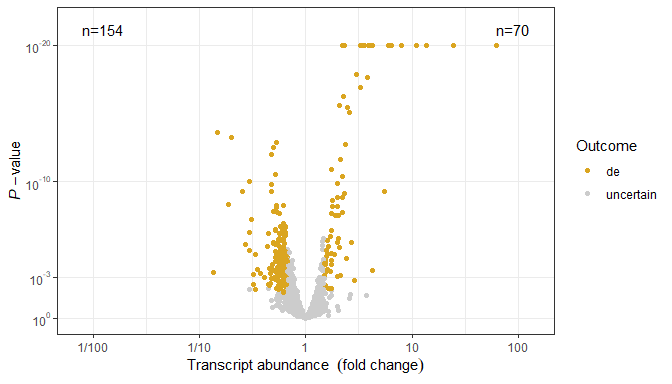# User Guide

## Preliminaries

We load all the packages used in the examples.

``````library(ggpmisc)
library(ggrepel)
library(xts)
library(lubridate)
library(tibble)
library(dplyr)
library(nlme)``````

As we will use text and labels on the plotting area we change the default theme to an uncluttered one.

``old_theme <- theme_set(theme_bw())``

## Introduction

Many of the functions, including ggplot statistics and geoms, included in package ‘ggpmisc’ had their origin in my need to produce plots for use in teaching or research reports. Some of them are generally useful, such as `stat_poly_eq()` and `stat_quadrant_counts()`but others like `stat_fit_deviations()` are squarely aimed and producing learning material. Function `try_tibble()` opens the door to easily converting time series stored in objects of several different classes into data frames. This is, for example, useful for plotting time series data with `ggplot()`. New `ggplot()` method specializations for classes `ts` and `xts` make the call to `try_tibble()` and conversion automatic.

## ggplot methods for time series

`ggplot()` methods for classes `"ts"` and `"xts"` automate plotting of time series data, as x and y aesthetics are mapped to time and the variable of the time series, respectively. For plotting time series data stored in objects of other classes, see the conversion functions `try_tibble()` and `try_data_frame()` in the last section of this vignette.

``class(lynx)``
``##  "ts"``
``ggplot(lynx) + geom_line()``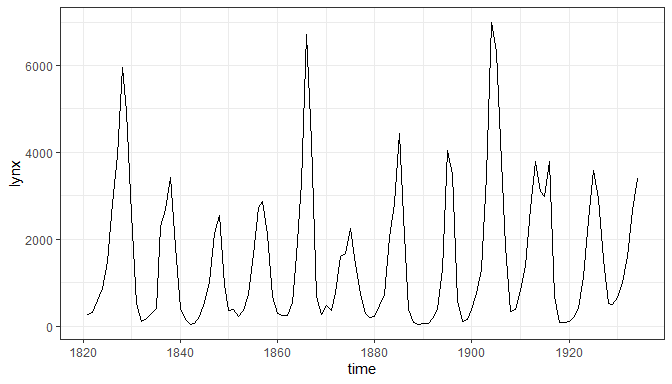It is possible to control the conversion of the time variable to numeric or datetime. As we will see below this affects the scale used by default as well as the formatting of values when converted to character strings or printed.

``ggplot(lynx, as.numeric = FALSE) + geom_line()``## Geometries

Three on the geometries described below allow the addition of plot layers containing insets. Insets can be plots, tables, bitmaps, or grid objects. Insets can be also added as annotations. Using for `data` a tibble with a list column containing data frames or tibbles allows like any other geom, the use of grouping, multiples insets per panel, faceting with different tables, and different number of insets in each panel, i.e., individual tables added to a plot with `geom_table` behave similarly to individual character values added with `geom_text`.

Other geometries also described in this section support the use of native plot coordinates for positioning elements in the plotting area. Obviously these geometries are not meant to display data, but instead to be able to add annotations to plots consistently across data sets and varying scales used.

### geom_table and stat_fmt_table

The geometry `geom_table()` plots a data frame or tibble, nested in a tibble passed as data argument, using aesthetics `x` and `y` for positioning, and `label` for the list of data frames containing the data for the tables. The tables are created as a ‘grid’ grobs and added as usual to the ggplot object. In contrast to “standard” geoms, this geom by default does not inherit the globally mapped aesthetics.

``````tb <- mpg %>%
group_by(cyl) %>%
summarise(hwy = median(hwy), cty = median(cty))

data.tb <- tibble(x = 7, y = 44, tb = list(tb))

ggplot(mpg, aes(displ, hwy, colour = factor(cyl))) +
geom_table(data = data.tb, aes(x, y, label = tb)) +
geom_point() ``````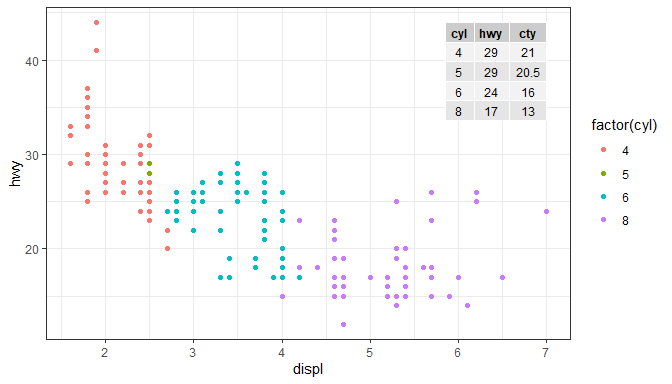In plots with a single panel it is easier to use `annotate()` to add inset tables, giving the same plot as above. In this case single data frames, ggplots or grobs do not need to be wrapped in a list, although lists are also supported.

``````tb <- mpg %>%
group_by(cyl) %>%
summarise(hwy = median(hwy), cty = median(cty))

ggplot(mpg, aes(displ, hwy, colour = factor(cyl))) +
annotate("table", x = 7, y = 44, label = tb) +
geom_point() ``````

Table themes are supported through parameter `table.theme` and if variables or constants are mapped to the `colour`, `fill`, `size`, `family`, aesthetics they override the corresponding theme settings. The display of `rownames` and `colnames` can be enabled or disable through parameter `table.rownames` and `table.colnames` and the horizontal justification of text in the core of the table through parameter `table.hjust`.

Parameter `table.theme` accepts as arguments `NULL` for use of the current default, a `ttheme` constructor function such as those defined in package ‘gridExtra’, or the variations on them defined in this package. The current default can be set with function `ttheme_set()`.

``````ggplot(mpg, aes(displ, hwy, colour = factor(cyl))) +
geom_table(data = data.tb, aes(x, y, label = tb),
table.theme = ttheme_gtsimple,
table.hjust = 0, colour = "darkred", fill = "#FFFFBB") +
geom_point() ``````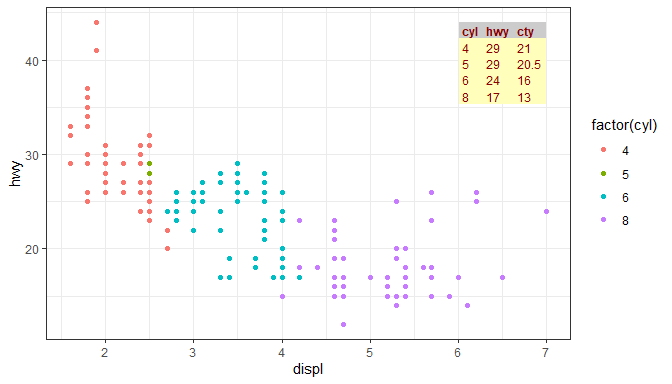Using `stat_fmt_tb()` we can rename columns and rows of the tibble, reorder them and/or select a subset of columns or rows as shown below. To provide a complete example we also replace the names of the scales for x, y and color aesthetics. Here we pass a `character` vector with the original names of the columns in full, but partial matching is tried when needed. It is also possible to use a `numeric` vector of positional indexes.

``````ggplot(mpg, aes(displ, hwy, colour = factor(cyl))) +
geom_table(data = data.tb, aes(x, y, label = tb),
table.theme = ttheme_gtlight,
size = 3, colour = "darkblue",
stat = "fmt_tb",
tb.vars = c(Cylinders = "cyl", MPG = "hwy"), # rename
tb.rows = 4:1) + # change order
labs(x = "Engine displacement (l)", y = "Fuel use efficiency (MPG)",
colour = "Engine cylinders\n(number)") +
geom_point() +
theme_bw()``````
``## Dropping column(s) from table.``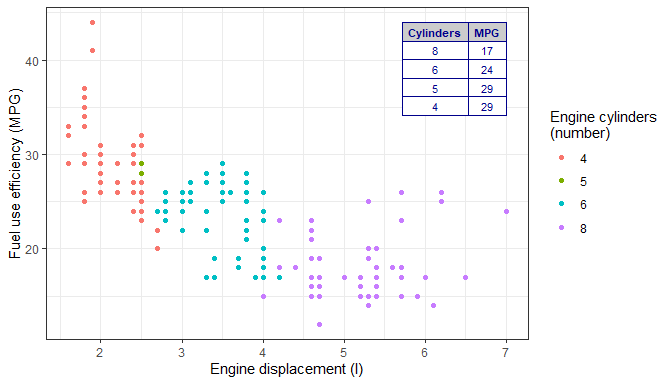Parsed text, using plot math syntax is supported in the table, with fall-back to plain text in case of parsing errors, on a cell by cell basis. Here we plot the MPG for city traffic and we can see that the plotting area expands to include the coordinates at which the table is anchored. Justification is by default set to `"inward"` which ensures that the table is fully within the plotting region.

``````tb.pm <- tibble(Parameter = c("frac(beta, a^2)", "frac(beta, a^3)"),
Value = c("10^2.4", "10^3.532"))
data.tb <- tibble(x = 7, y = 44, tb = list(tb.pm))
ggplot(mpg, aes(displ, cty)) +
geom_point() +
geom_table(data = data.tb, aes(x, y, label = tb), parse = TRUE) +
theme_bw()``````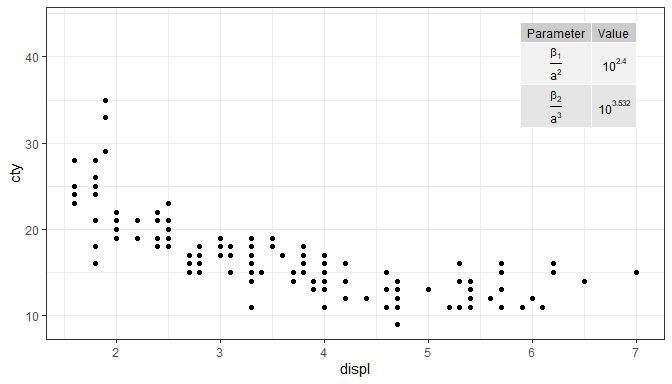As implemented, there is no limitation to the number of insets, and faceting is respected. If the base plot shows a map, multiple small tables could be superimposed on different countries or regions. The size of the insets is set relative to the main plot, so the combined plot can be scaled.

Please see section Normalised Parent Coordinates below for a description of `geom_table_npc()`.

### geom_plot

The `geom_plot()` geometry plots ggplot objects, nested in a tibble passed as data argument, using aesthetics `x` and `y` for positioning, and `label` for the ggplot object containing the definition of the plot to be nested. With this approach in plots with facets the insets can be different in each panel. It is also possible to inset more than one plot in a single call simply by creating a tibble with multiple rows.

Behind the scenes, one Grob is created for each plot to be inset. The conversion is done with `ggplotGrob()` and the Grobs added the main ggplot object.

As an example we produce a plot where the inset plot is a zoomed-in detail from the main plot. In this case the main and inset plots start as the same plot. In most cases the size of text and other elements in the inset should be smaller than in the main plot. Here we override the default theme setting the `base_size` from its default of 11 pt to 8 pt.

``````p <- ggplot(mpg, aes(displ, hwy, colour = factor(cyl))) +
geom_point()

data.tb <-
tibble(x = 7, y = 44,
plot = list(p +
coord_cartesian(xlim = c(4.9, 6.2),
ylim = c(13, 21)) +
labs(x = NULL, y = NULL) +
theme_bw(8) +
scale_colour_discrete(guide = FALSE)))

ggplot(mpg, aes(displ, hwy, colour = factor(cyl))) +
geom_plot(data = data.tb, aes(x, y, label = plot)) +
annotate(geom = "rect",
xmin = 4.9, xmax = 6.2, ymin = 13, ymax = 21,
linetype = "dotted", fill = NA, colour = "black") +
geom_point() ``````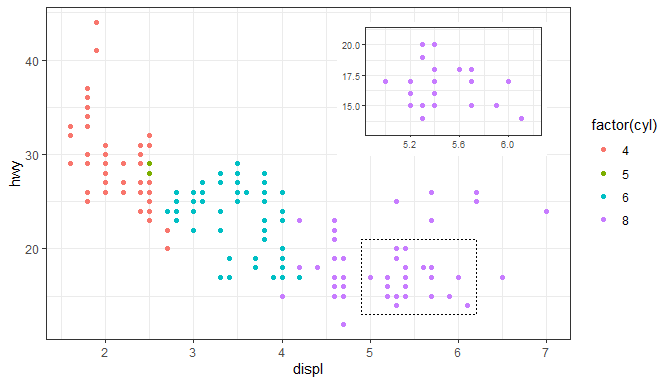In general, the inset plot can be any ggplot object, allowing the creation of very different combinations of main plot and inset plots. Here we use the inset to show summaries as in the previous example of an inset table.

``````p <- ggplot(mpg, aes(factor(cyl), hwy, fill = factor(cyl))) +
stat_summary(geom = "col", fun = mean, width = 2/3) +
labs(x = "Number of cylinders", y = NULL, title = "Means") +
scale_fill_discrete(guide = FALSE)

data.tb <- tibble(x = 7, y = 44,
plot = list(p +
theme_bw(8)))

ggplot(mpg, aes(displ, hwy, colour = factor(cyl))) +
geom_plot(data = data.tb, aes(x, y, label = plot)) +
geom_point() +
labs(x = "Engine displacement (l)", y = "Fuel use efficiency (MPG)",
colour = "Engine cylinders\n(number)") +
theme_bw()``````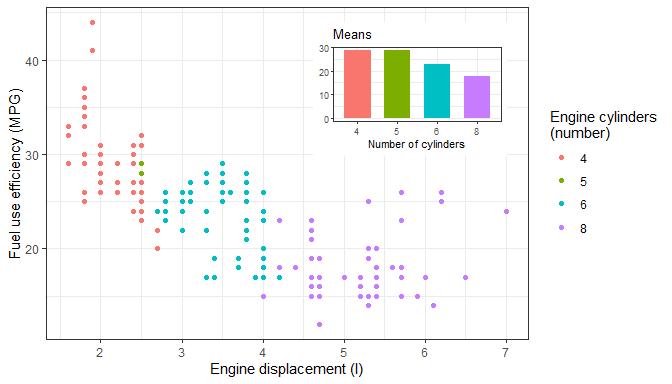The same plot as above can be created using `annotate()` but be aware that when using facets in ‘ggplot2’ annotations are identical in all panels.

``````p <- ggplot(mpg, aes(factor(cyl), hwy, fill = factor(cyl))) +
stat_summary(geom = "col", fun = mean, width = 2/3) +
labs(x = "Number of cylinders", y = NULL, title = "Means") +
scale_fill_discrete(guide = FALSE)

ggplot(mpg, aes(displ, hwy, colour = factor(cyl))) +
annotate("plot", x = 7, y = 44, label = p + theme_bw(8)) +
geom_point() +
labs(x = "Engine displacement (l)", y = "Fuel use efficiency (MPG)",
colour = "Engine cylinders\n(number)") +
theme_bw()``````

As implemented, there is no limitation to the number of insets, and faceting is respected. If the base plot shows a map or a bitmap, multiple small plots could be superimposed on different countries or regions. The size of the insets is set relative to the main plot, so the combined plot can be scaled. A possible unintuitive but useful feature, is that the theme is linked to each plot.

Please see section Normalised Parent Coordinates below for a description of `geom_plot_npc()`.

### geom_grob

The `geom_grob()` geometry plots grobs (graphical objects as created with ‘grid’), nested in a tibble passed as data argument, using aesthetics `x` and `y` for positioning, and `label` for the Grob object. While `geom_table()` and `geom_plot()` take as values to plot tibbles or data frames, and ggplots, respectively, and convert them into Grobs before adding them to the plot, `geom_grob()` expects Grobs ready to be rendered.

``````file.name <-
system.file("extdata", "Isoquercitin.png",
package = "ggpmisc", mustWork = TRUE)
grobs.tb <- tibble(x = c(0, 10, 20, 40), y = c(4, 5, 6, 9),
width = c(0.05, 0.05, 0.01, 1),
height =  c(0.05, 0.05, 0.01, 0.3),
grob = list(grid::circleGrob(),
grid::rectGrob(),
grid::textGrob("I am a Grob"),
grid::rasterGrob(image = Isoquercitin)))

ggplot() +
geom_grob(data = grobs.tb,
aes(x, y, label = grob, vp.width = width, vp.height = height),
hjust = 0.7, vjust = 0.55) +
scale_y_continuous(expand = expansion(mult = 0.3, add = 0)) +
scale_x_continuous(expand = expansion(mult = 0.2, add = 0)) +
theme_bw(12)``````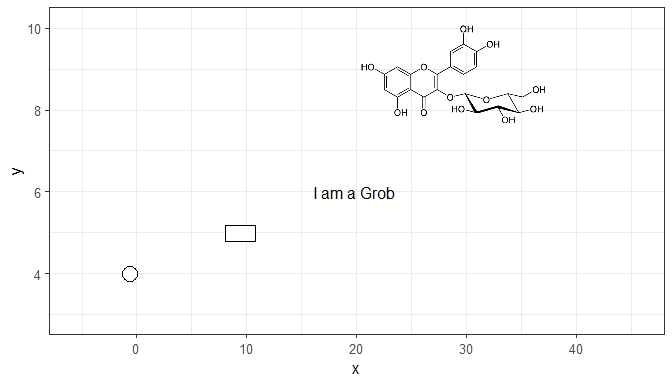As shown above for inset tables and inset plots, it is also possible to use `annotate()` with Grobs. The next example insets a single `Grob`. Here we reuse the bitmap `Isoquercitin` read in the previous example. The Grob is contained in a viewport. Here setting `width = 1` (“npc” units) when creating the `Grob` from the bitmap ensures that the bitmap fills the width of the viewport (to ensure that the inset is not distorted, set only one of `width` or `height`). The argument to `vp.width` or `vp.height`, also in “npc” units, determines the size of the `Grob` relative to the size of the plotting area.

``````ggplot() +
annotate("grob", x = 1, y = 3, vp.width = 0.5,
label = grid::rasterGrob(image = Isoquercitin, width = 1)) +
theme_bw(12)``````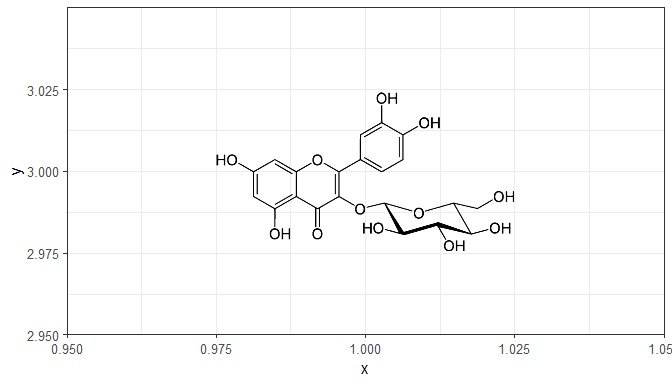`geom_grob()` is designed thinking that its main use will in graphical annotations, although one could use it for infographics with multiple copies of each grob, this would go against the grammar of graphics. In this implementation grobs cannot be mapped to an aesthetic through a scale.

As implemented, there is no limitation to the number of insets and faceting is respected. If the base plot shows a map or a bitmap, multiple simple grobs (e.g. national flags) could be superimposed on different countries. The size of the insets is set relative to the main plot, so the combined plot can be scaled.

Please see section Normalised Parent Coordinates below for a description of `geom_grob_npc()`.

### geom_vhlines

This is a convenience geometry that adds both vertical and horizontal guide lines on the same plot layer, using the same syntax as `geom_hline()` and `geom_vline()` from package ‘ggplot2’.

``````ggplot(mpg, aes(displ, hwy, colour = factor(cyl))) +
geom_vhlines(xintercept = c(2.75, 4), yintercept = 27, linetype = "dashed") +
geom_point() +
labs(x = "Engine displacement (l)", y = "Fuel use efficiency (MPG)",
colour = "Engine cylinders\n(number)") +
theme_bw()``````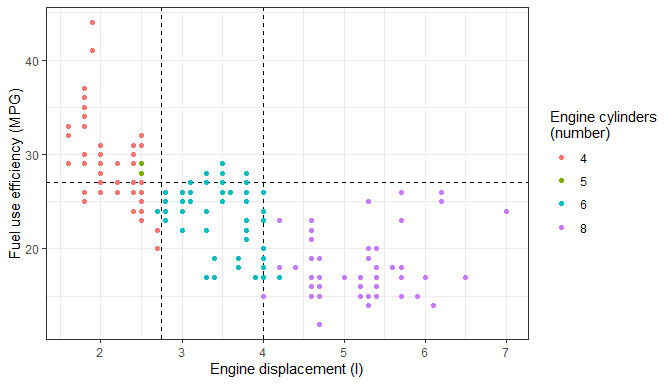### Normalised Parent Coordinates

R’s ‘grid’ package defines several units that can be used to describe the locations of plot elements. In ‘ggplot2’ the x and y aesthetics are directly mapped to `"native"` or data units. For consistent location of annotations with respect to the plotting area we need to rely on `"npc"` which are expressed relative to the size of the grid viewport. The plotting area in a ggplot is implemented as viewport and support for `"npc"` coordinates is relatively easy to implement.

To support `"npc"` positions we have implemented scales for two new aesthetics, `npcx` and `npcy`. These are very simple continuous scales which do not support any transformation or changes to their limits, both of which would be meaningless for `"npc"` units. Variables mapped to these aesthetics can be either numerical with values in the range zero to one or character. A limited set of strings are recognised and converted to `"npc"` units: `"bottom"`, `"center"`, `"top"`, `"left"`, `"middle"`, `"right"` (`"centre"` is a synonym for `"center"`).

To make these scales useful we need also to define geometries that use these new aesthetics. Package ‘ggpmisc’ currently provides `geom_text_npc()`, `geom_label_npc()`, `geom_table_npc()`, `geom_plot_npc()` and `geom_grob_npc()`.

As is the case for `geom_text()` and `geom_label()` from package ‘ggplot2’, `"bottom"`, `"center"`, `"top"`, `"left"`, `"middle"`, `"right"`, plus `"inward"` and `"outward"` can be used, as well as numeric values, to control the justification. Justification defaults to `"inward"` in the geometries described here.

While the usual x and y aesthetics are used whenever the positions of plot elements represent data values, these new scales and geometries are useful only for annotations, i.e., in those cases when we want plot elements at specific positions within the plotting area irrespective of the ranges of the data mapped to the x and y aesthetics. When writing scripts or functions that may be applied to different data sets these new aesthetics help by keeping the code concise and reusable. These geometries are used by default by several of the statistics described in later sections.

As an example let’s imagine that we want to add the structure of a metabolite to a plot. Its position has nothing to do with the data mapped to x and y, so it is conceptually better to use `"npc"` coordinates. The big practical advantage is that this also allows to keep this part of the plot definition independent of the data being plotted, giving a major advantage in the case of plots with facets with free scale limits. This example can be easily adapted to `geom_plot_npc()` where a ggplot should mapped to `label`, and to `geom_table_npc()` where a data frame should be mapped to `label`.

We produce the example plot by first constructing a tibble to contain the grob and the coordinate data, and then map these variables to aesthetics using `aes()`. In the example the tibble has a single row, but this is not a requirement. In this respect these geoms behave as normal geoms, with facets also supported.

``````file.name <-
system.file("extdata", "Robinin.png",
package = "ggpmisc", mustWork = TRUE)

set.seed(123456)
data.tb <- tibble(x = 1:20, y = (1:20) + rnorm(20, 0, 10))

flavo.tb <- tibble(x = 0.02,
y = 0.95,
width = 1/2,
height = 1/4,
grob = list(grid::rasterGrob(image = Robinin)))

ggplot(data.tb, aes(x, y)) +
geom_grob_npc(data = flavo.tb,
aes(label = grob, npcx = x, npcy = y,
vp.width = width, vp.height = height)) +
geom_point() +
expand_limits(y = 55, x = 0)``````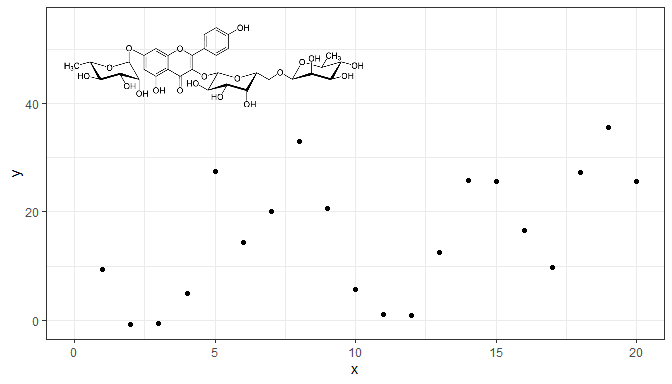Alternatively, we can pass constant values to `geom_grob_npc()` to obtain the same plot. This approach can be handy in simple cases.

``````ggplot(data.tb, aes(x, y)) +
geom_grob_npc(label = list(grid::rasterGrob(image = Robinin, width = 1)),
npcx = 0.02, npcy = 0.95,
vp.width = 1/2, vp.height = 1/4) +
geom_point() +
expand_limits(y = 55, x = 0)``````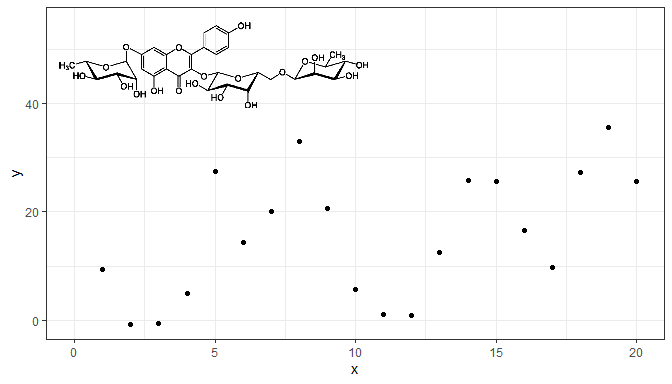We can also use `annotate()` if the annotation should be the same for all panels, or if we have a single figure panel. In this case there is no need to wrap a single `grob` in a list.

``````ggplot(data.tb, aes(x, y)) +
annotate("grob_npc", label = grid::rasterGrob(image = Robinin, width = 1),
npcx = 0.02, npcy = 0.95, vp.width = 1/2, vp.height = 1/4) +
geom_point() +
expand_limits(y = 55, x = 0)``````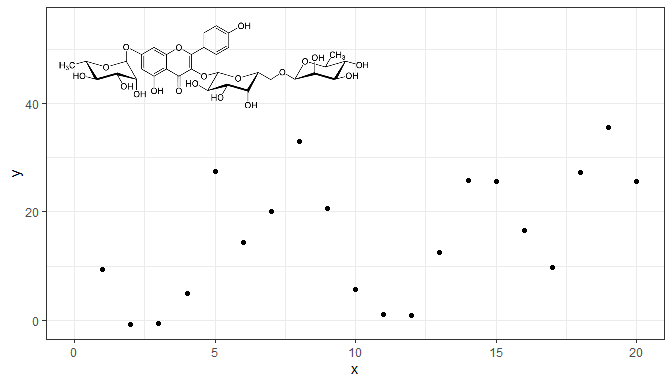Two additional geometries are based on existing ‘ggplot2’ geometries. They are based on `geom_text()` and `geom_label()`. We give an example using `geom_text_npc()` to produce a “classic” labelling for facets matching the style of `theme_classic()` and traditional scientific journals’ design.

``````corner_letters.tb <- tibble(label = LETTERS[1:4],
x = "left",
y = "top",
cyl = c(4,5,6,8))
ggplot(mpg, aes(displ, hwy)) +
geom_point() +
facet_wrap(~cyl, scales = "free") +
geom_text_npc(data = corner_letters.tb,
aes(npcx = x, npcy = y, label = label)) +
theme_classic() +
theme(strip.background = element_blank(),
strip.text.x = element_blank())``````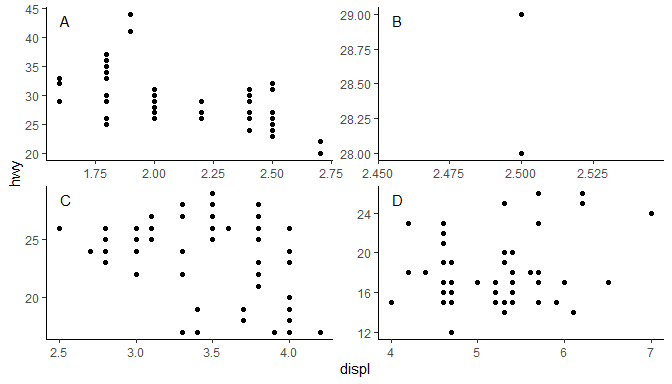### Marginal markings

‘ggplot2’ provides `geom_rug()`, `geom_vline()` and `geom_hline()`. Rug plots are intended to be used to represent distributions along the margins of plot. `geom_vline()` and `geom_hline()`are normally used to separate regions in a plot or to highlight important values along the x or y axis. When creating plots it is sometimes useful to put small marks along the axes, just inside the plotting area, similar to those in a rug plot, but like `geom_vline()` and `geom_hline()` in their purpose.

Three geometries provide such markers: `geom_margin_point()`, `geom_margin_arrow()`, and `geom_margin_grob()`. They behave similarly to `geom_vline()` and `geom_hline()` and their positions are determined also by the `xintercept` and `yintercept` aesthetics.

In the example below we indicate the group medians along the x axis with filled triangles.

``````data.tb <- mpg %>%
group_by(cyl) %>%
summarise(hwy = median(hwy), displ = median(displ))
ggplot(mpg, aes(displ, hwy, colour = factor(cyl))) +
geom_x_margin_point(data = data.tb,
aes(xintercept = displ, fill = factor(cyl))) +
expand_limits(y = 10) +
geom_point() ``````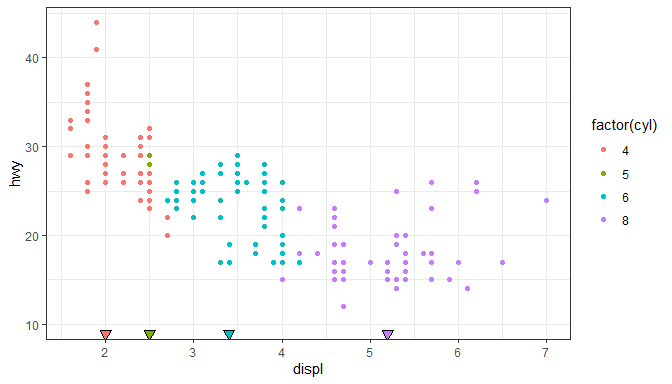## Statistics

### stat_centroid

It can be useful to mark the centroid of a group of observations with a point or with a label. By default `stat_centroid()` applies function `mean()` to both `x` and `y` by group. If all the values mapped to `label` within each group are identical, this value is copied to the returned `data`.

``````ggplot(mpg, aes(displ, hwy, colour = factor(cyl))) +
geom_point(alpha = 0.33) +
stat_centroid(shape = "cross", size = 4)``````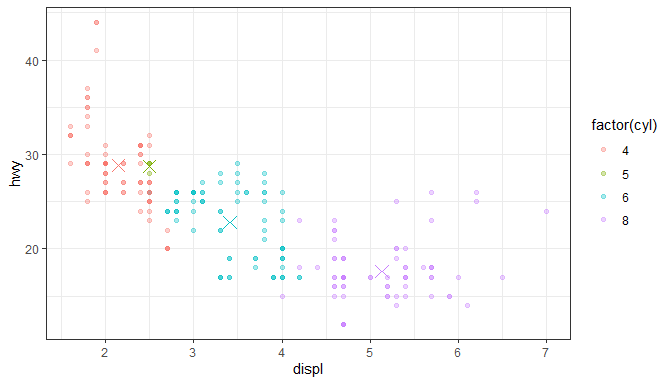Other functions can be passed to this statistic as long as they return a single value that can be mapped to the `x` and `y` aesthetics (numeric, time or a factor).

``````ggplot(mpg, aes(displ, hwy, colour = factor(cyl))) +
geom_point(alpha = 0.33) +
stat_centroid(shape = "cross", size = 4, .fun = median)``````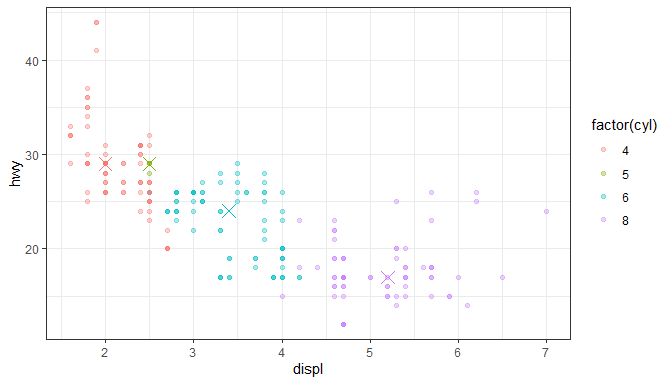The very similar `stat_summary_xy()` accepts different functions for `x` and `y`.

### stat_peaks and stat_valleys

Two statistics make it possible to highlight and/or label peaks and valleys (local maxima and local minima) in a curve. Here we use a time series, using POSIXct for `time` and the default formatting of labels. We also instruct `geom_text()` not to render overlapping labels.

``````ggplot(lynx, as.numeric = FALSE) + geom_line() +
stat_peaks(colour = "red") +
stat_peaks(geom = "text", colour = "red", vjust = -0.5,
check_overlap = TRUE) +
ylim(-100, 7300)``````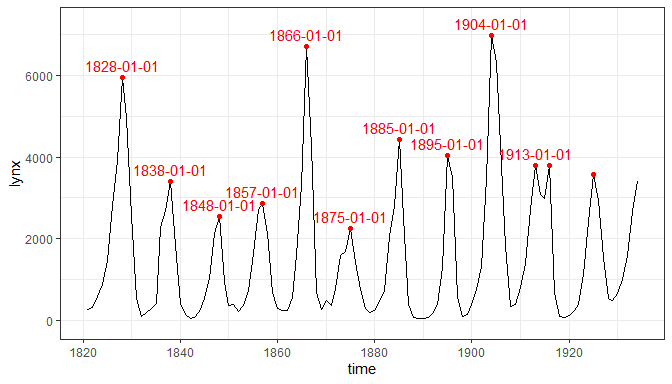Using numeric values for `time` and the default formatting of labels. We can also pass other aesthetics recognised the `geom_text()` such as `angle`. Here we also highlight and label the valleys.

``````ggplot(lynx) + geom_line() +
stat_peaks(colour = "red") +
stat_peaks(geom = "text", colour = "red", hjust = -0.2, vjust = 0.5,
angle = 90, check_overlap = TRUE) +
stat_valleys(colour = "blue") +
stat_valleys(geom = "text", colour = "blue", hjust = 1.2, vjust = 0.5,
angle = 90, check_overlap = TRUE) +
expand_limits(y = c(-900, 8000))``````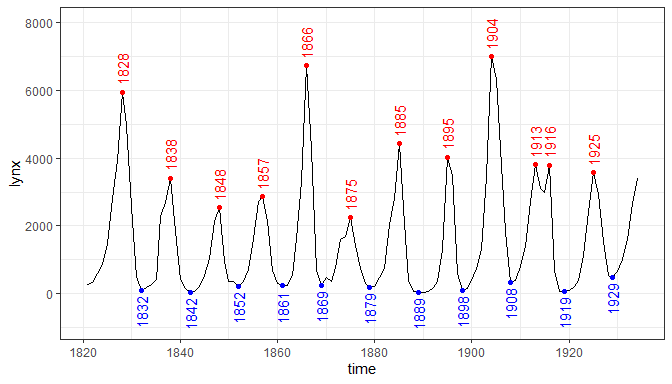Using POSIXct for `time` but supplying a format string. In addition marking both peaks and valleys.

``````ggplot(lynx, as.numeric = FALSE) + geom_line() +
stat_peaks(colour = "red") +
stat_peaks(geom = "text", colour = "red", hjust = -0.2, vjust = 0.5,
angle = 90, check_overlap = TRUE, x.label.fmt = "%Y") +
stat_valleys(colour = "blue") +
stat_valleys(geom = "text", colour = "blue", hjust = 1.2, vjust = 0.5,
angle = 90, check_overlap = TRUE, x.label.fmt = "%Y") +
expand_limits(y = c(-900, 8000))``````Using `geom_rug` for the peaks and valleys.

``````ggplot(lynx, as.numeric = FALSE) + geom_line() +
stat_peaks(colour = "red") +
stat_peaks(geom = "rug", colour = "red") +
stat_valleys(colour = "blue") +
stat_valleys(geom = "rug", colour = "blue")``````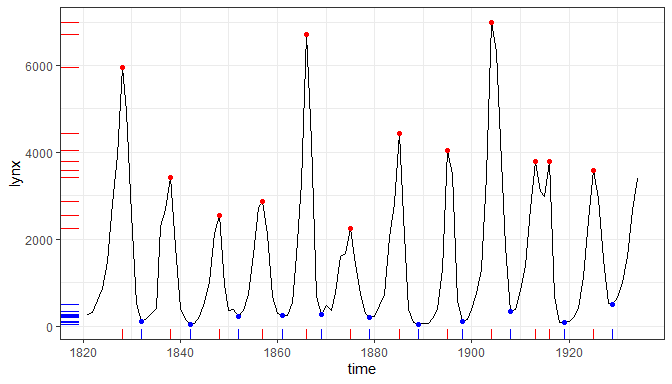This statistic automates the annotation of plots with number of observations, either by quadrant, by pairs of quadrants or the four quadrants taken together (whole plotting area). Its companion geometry, `geom_quadrant_lines()` is used in the examples to highlight the quadrants.

We generate some artificial data.

``````set.seed(4321)
# generate artificial data
x <- -99:100
y <- x + rnorm(length(x), mean = 0, sd = abs(x))
my.data <- data.frame(x,
y,
group = c("A", "B"))``````

Using defaults except for color.

``````ggplot(my.data, aes(x, y)) +
geom_point() +
expand_limits(y = c(-250, 250))``````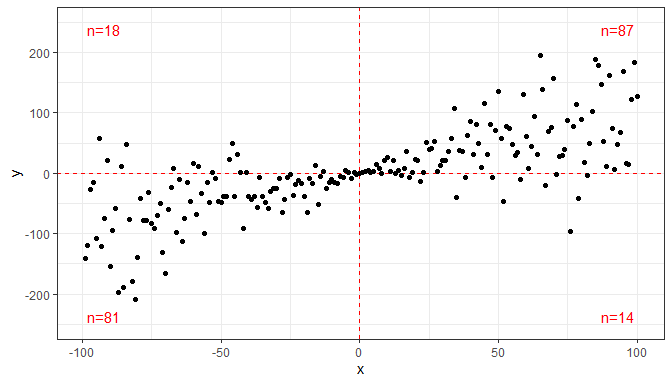Pooling quadrants along the x-axis. (`pool.along = "y"` pools along y.)

``````ggplot(my.data, aes(x, y)) +
geom_quadrant_lines(colour = "red", pool.along = "x") +
stat_quadrant_counts(colour = "red", pool.along = "x") +
geom_point()``````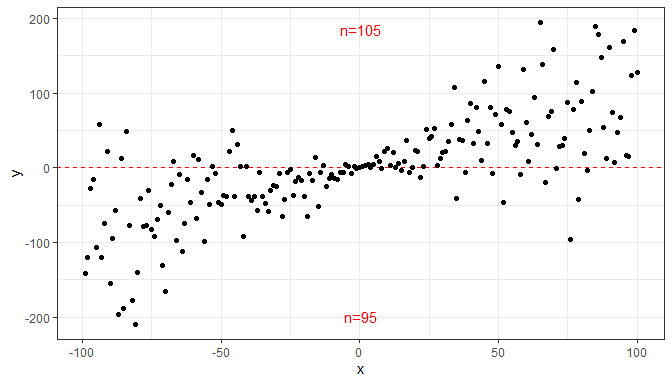Manual positioning of the text annotations and pooling of all four quadrants, and overriding the default formatting for the label.

``````ggplot(my.data, aes(x, y)) +
geom_point() +
aes(label = sprintf("%i observations", stat(count))))``````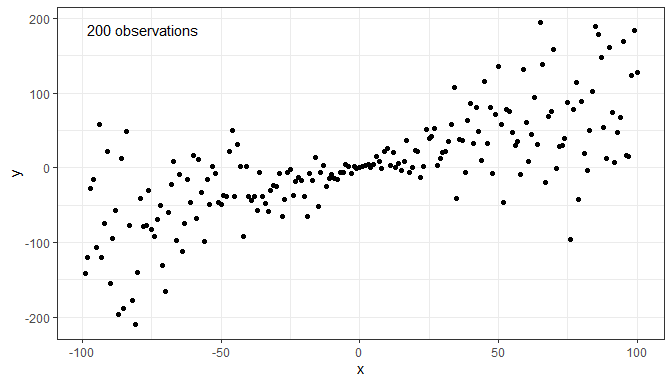``````ggplot(my.data, aes(x, y)) +
geom_point()``````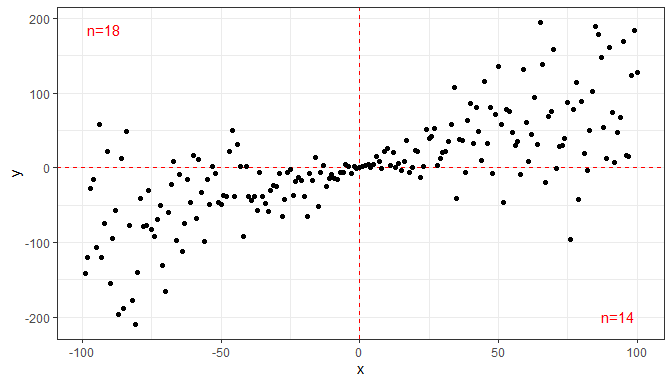Using facets, even with free scale limits, the labels are placed consistently. This achieved by the default use of `geom_text_npc()` or as shown below by use of `geom_label_npc(). We expand the y limits to ensure that no observations are occluded by the labels.

``````ggplot(my.data, aes(x, y, colour = group)) +
geom_point() +
expand_limits(y = c(-260, 260)) +
facet_wrap(~group)``````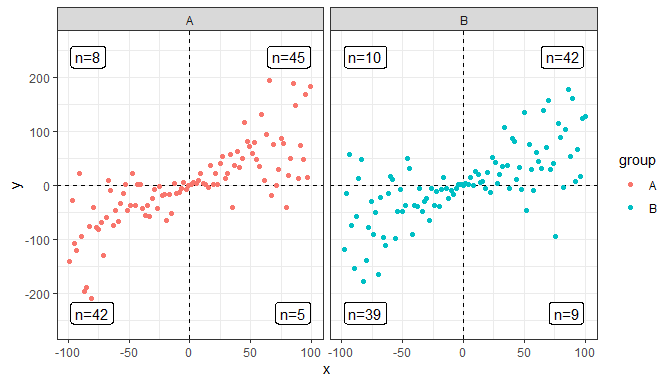### stat_poly_eq

A frequently used annotation in plots showing fitted lines is the display of the parameters estimates from the model fit in an equation. `stat_poly_eq` automates this for linear model of y on x fitted with function `lm`. This statistic behaves similarly as `ggplot2::stat_smooth` with `method = "lm"`. Other statistics described in later sections allow various annotations based on different model fit methods but require additional effort from the user. The default behaviour is to generate labels as character strings using expression syntax. This stat can also output equations using different markup languages, including \(\LaTeX\) and Markdown, selected by the argument passed to parameter `output.type`. Nevertheless, all examples below use expression syntax.

We generate a set of artificial data suitable for the next examples.

``````set.seed(4321)
# generate artificial data
x <- 1:100
y <- (x + x^2 + x^3) + rnorm(length(x), mean = 0, sd = mean(x^3) / 4)
my.data <- data.frame(x,
y,
group = c("A", "B"),
y2 = y * c(0.5,2),
block = c("a", "a", "b", "b"),
wt = sqrt(x))``````

First one example using defaults. The best practice, ensuring that the formula used in both statistics is the same is assign the formula to a variable, as shown here. This is because the same model is fit twice to the same `data`, once in `stat_smooth` and once in `stat_poly_eq`.

``````formula <- y ~ poly(x, 3, raw = TRUE)
ggplot(my.data, aes(x, y)) +
geom_point() +
stat_smooth(method = "lm", formula = formula) +
stat_poly_eq(formula = formula, parse = TRUE)``````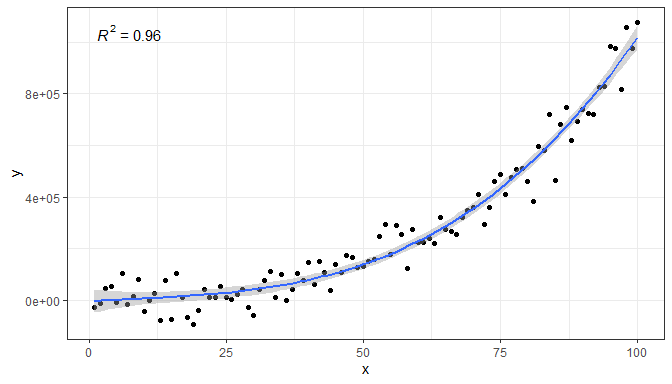A ready formatted equation is also returned as a string that needs to be parsed into an expression for display.

``````formula <- y ~ poly(x, 3, raw = TRUE)
ggplot(my.data, aes(x, y)) +
geom_point() +
geom_smooth(method = "lm", formula = formula) +
stat_poly_eq(aes(label = stat(eq.label)), formula = formula,
parse = TRUE)``````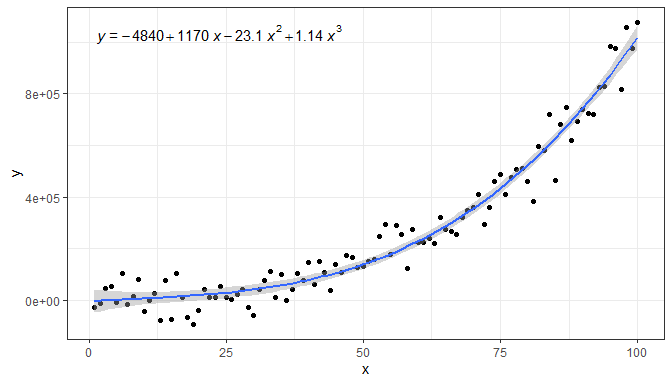`stat_poly_eq()` makes available several character strings in the returned data frame in separate columns: `eq.label`, `rr.label`, `adj.rr.label`, `AIC.label`, `BIC.label`, `f.value.label` and `p.value.label`. One of these, `rr.label`, is used by default, but `aes()` can be used to map a different one to the `label` aesthetic. Here we show the adjusted coefficient of determination and AIC. We call `stat_poly_eq()` twice to be able to separately control the position and size of each label.

``````formula <- y ~ poly(x, 3, raw = TRUE)
ggplot(my.data, aes(x, y)) +
geom_point() +
geom_smooth(method = "lm", formula = formula) +
stat_poly_eq(aes(label = stat(adj.rr.label)), formula = formula,
parse = TRUE) +
stat_poly_eq(aes(label = stat(AIC.label)),
label.x = "right", label.y = "bottom", size = 3,
formula = formula,
parse = TRUE)``````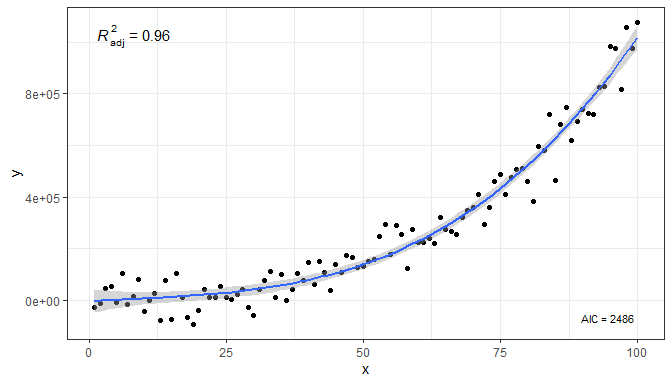Within `aes()` it is also possible to compute new labels based on those returned plus “arbitrary” text. The labels returned by default are meant to be parsed into expressions, so any text added should be valid for a string that will be parsed. Inserting a comma plus white space in an expression requires some trickery in the argument passed to `sep`. Do note the need to escape the embedded quotation marks as `\"`. Combining the labels in this way ensures correct alignment. To insert only white space `sep = "~~~~~"` can be used, with each tilde character, `~`, adding a rather small amount of white space. We show only here, not to clutter the remaining examples, how to change the axis labels to ensure consistency with the typesetting of the equation.

``````formula <- y ~ poly(x, 3, raw = TRUE)
ggplot(my.data, aes(x, y)) +
geom_point() +
geom_smooth(method = "lm", formula = formula) +
sep = "*\", \"*")),
formula = formula, parse = TRUE) +
labs(x = expression(italic(x)), y = expression(italic(y)))``````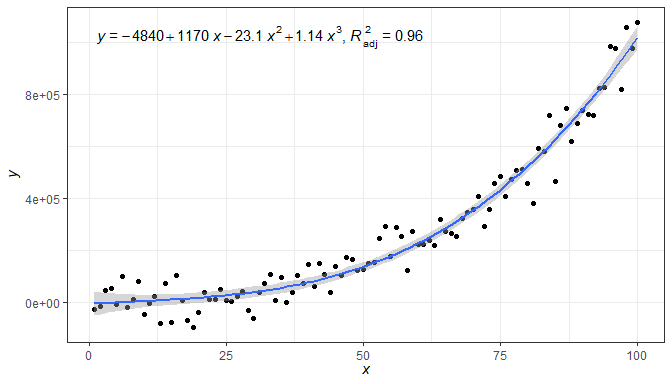Above we combined two character-string labels, but it is possible to add additional ones and even character strings constants. In this example we use several labels instead of just two, and separate them with various character strings. We also change the size of the text in the label.

``````formula <- y ~ poly(x, 3, raw = TRUE)
ggplot(my.data, aes(x, y)) +
geom_point() +
geom_smooth(method = "lm", formula = formula) +
stat_poly_eq(aes(label =  paste(stat(eq.label), "*\" with \"*",
stat(rr.label), "*\", \"*",
stat(f.value.label), "*\", and \"*",
stat(p.value.label), "*\".\"",
sep = "")),
formula = formula, parse = TRUE, size = 3)``````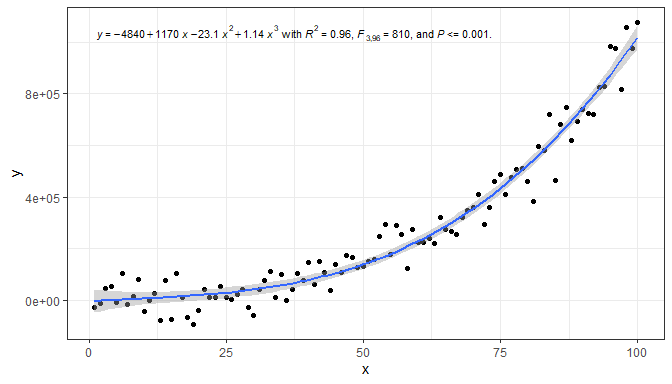It is also possible to format the text using `plain()`, `italic()`, `bold()` or `bolditalic()` as described in `plotmath`.

``````formula <- y ~ poly(x, 3, raw = TRUE)
ggplot(my.data, aes(x, y)) +
geom_point() +
geom_smooth(method = "lm", formula = formula) +
sep = "~~italic(\"with\")~~")),
formula = formula, parse = TRUE)``````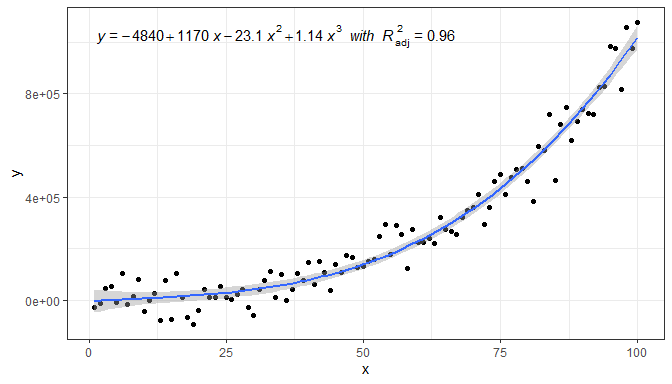As these are expressions, to include two lines of text, we need either to add `stat_poly_eq()` twice, passing an argument to `label.y` to reposition one of the labels (as shown above) or use (as shown here) `atop()` within the expression to create a single label with two lines of text.

``````formula <- y ~ poly(x, 3, raw = TRUE)
ggplot(my.data, aes(x, y)) +
geom_point() +
geom_smooth(method = "lm", formula = formula) +
stat_poly_eq(aes(label = paste("atop(", stat(AIC.label), ",", stat(BIC.label), ")", sep = "")),
formula = formula,
parse = TRUE)``````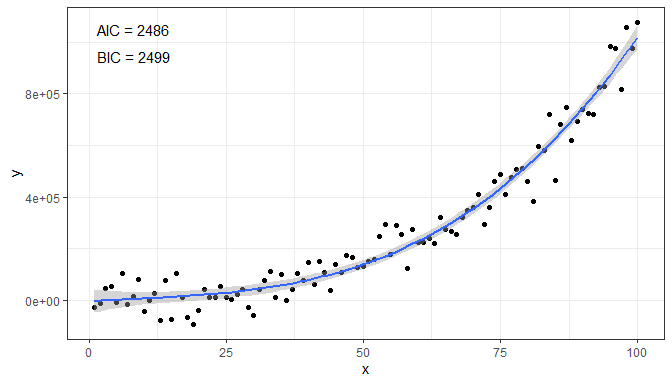Next, one example of how to remove the left hand side (lhs).

``````formula <- y ~ poly(x, 3, raw = TRUE)
ggplot(my.data, aes(x, y)) +
geom_point() +
geom_smooth(method = "lm", formula = formula) +
stat_poly_eq(aes(label = stat(eq.label)),
eq.with.lhs = FALSE,
formula = formula, parse = TRUE)``````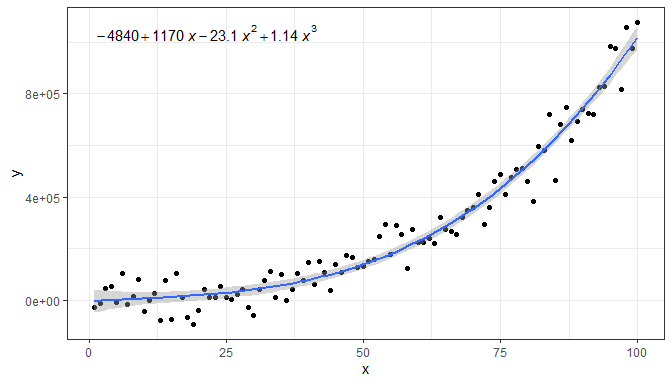Replacing the lhs.

``````formula <- y ~ poly(x, 3, raw = TRUE)
ggplot(my.data, aes(x, y)) +
geom_point() +
geom_smooth(method = "lm", formula = formula) +
stat_poly_eq(aes(label = stat(eq.label)),
eq.with.lhs = "italic(hat(y))~`=`~",
formula = formula, parse = TRUE)``````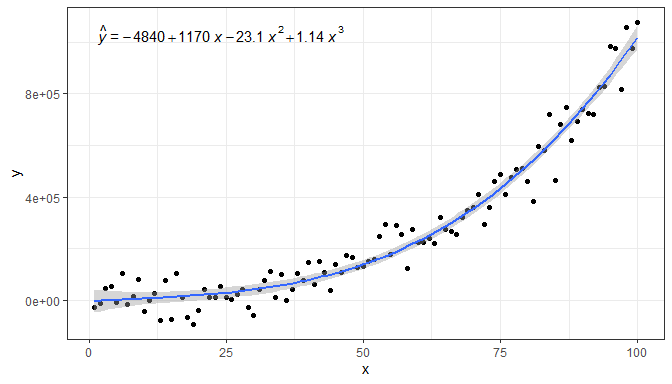And a final example replacing both the lhs and the variable symbol used on the rhs.

``````formula <- y ~ poly(x, 3, raw = TRUE)
ggplot(my.data, aes(x, y)) +
geom_point() +
geom_smooth(method = "lm", formula = formula) +
labs(x = expression(italic(z)), y = expression(italic(h)) ) +
stat_poly_eq(aes(label = stat(eq.label)),
eq.with.lhs = "italic(h)~`=`~",
eq.x.rhs = "~italic(z)",
formula = formula, parse = TRUE)``````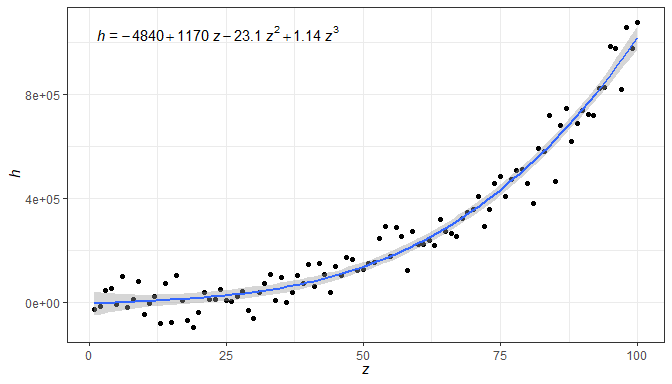As any valid R expression can be used, Greek letters are also supported, as well as the inclusion in the label of variable transformations used in the model formula or applied to the data. In addition, in the next example we insert white space in between the parameter estimates and the variable symbol in the equation.

``````formula <- y ~ poly(x, 2, raw = TRUE)
ggplot(my.data, aes(x, log10(y + 1e6))) +
geom_point() +
geom_smooth(method = "lm", formula = formula) +
stat_poly_eq(aes(label = stat(eq.label)),
eq.with.lhs = "plain(log)(italic(delta)+10^6)~`=`~",
eq.x.rhs = "~Omega",
formula = formula, parse = TRUE) +
labs(y = expression(plain(log)(italic(delta)+10^6)), x = expression(Omega))``````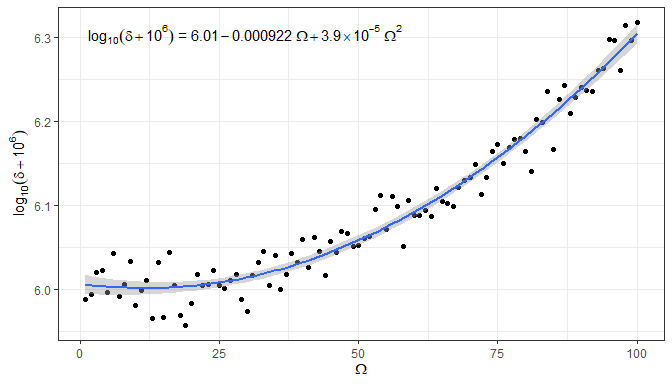A couple of additional examples of polynomials of different orders, and specified in different ways.

Higher order polynomial.

``````formula <- y ~ poly(x, 5, raw = TRUE)
ggplot(my.data, aes(x, y)) +
geom_point() +
geom_smooth(method = "lm", formula = formula) +
stat_poly_eq(aes(label = stat(eq.label)), formula = formula, parse = TRUE)``````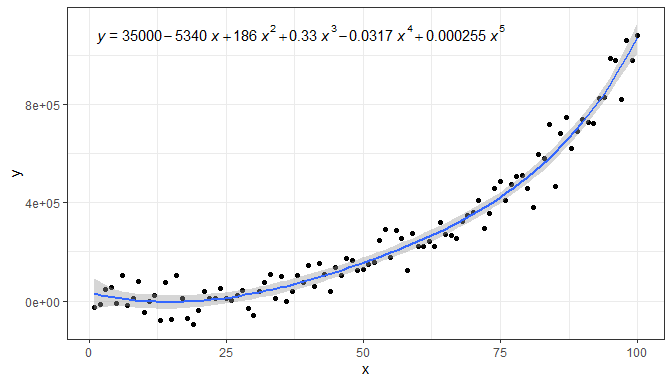Intercept forced to zero.

``````formula <- y ~ x + I(x^2) + I(x^3) - 1
ggplot(my.data, aes(x, y)) +
geom_point() +
geom_smooth(method = "lm", formula = formula) +
stat_poly_eq(aes(label = stat(eq.label)), formula = formula,
parse = TRUE)``````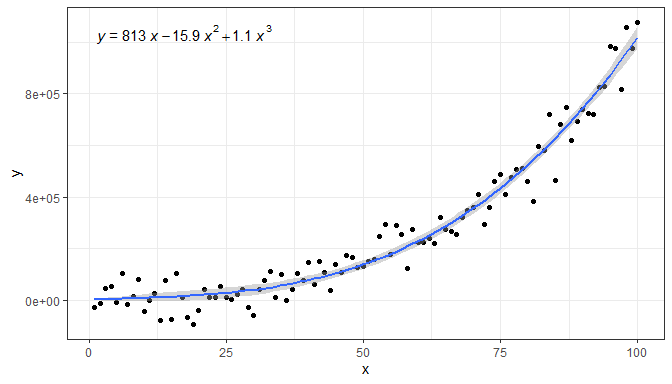We give below several examples to demonstrate how other components of the `ggplot` object affect the behaviour of this statistic.

Facets work as expected either with fixed or free scales. Although below we had to adjust the size of the font used for the equation.

``````formula <- y ~ poly(x, 3, raw = TRUE)
ggplot(my.data, aes(x, y2)) +
geom_point() +
geom_smooth(method = "lm", formula = formula) +
stat_poly_eq(aes(label = stat(eq.label)), size = 3,
formula = formula, parse = TRUE) +
facet_wrap(~group)``````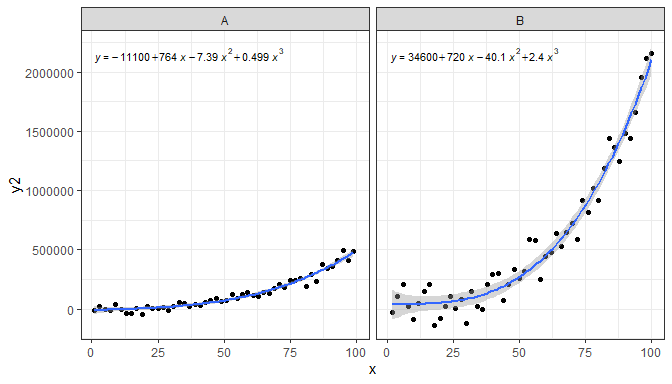``````formula <- y ~ poly(x, 3, raw = TRUE)
ggplot(my.data, aes(x, y2)) +
geom_point() +
geom_smooth(method = "lm", formula = formula) +
stat_poly_eq(aes(label = stat(eq.label)), size = 3,
formula = formula, parse = TRUE) +
facet_wrap(~group, scales = "free_y")``````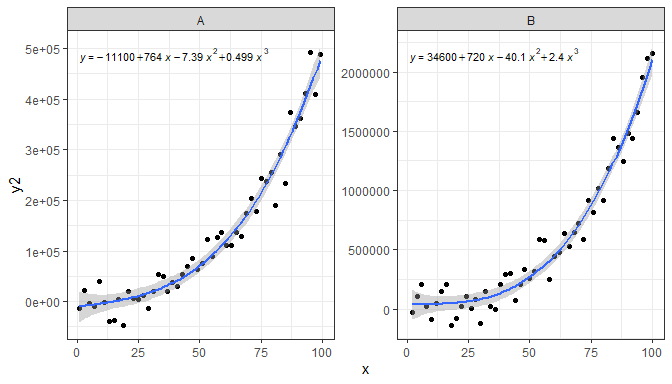Grouping, in this example using the color aesthetic also works as expected. We can use justification and supply an absolute location for the equation, but the default frequently works well as in the example below.

``````formula <- y ~ poly(x, 3, raw = TRUE)
ggplot(my.data, aes(x, y2, colour = group)) +
geom_point() +
geom_smooth(method = "lm", formula = formula) +
stat_poly_eq(aes(label = stat(eq.label)),
formula = formula, parse = TRUE)``````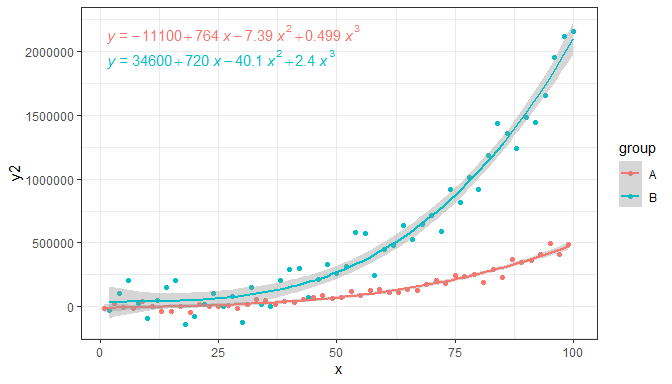To add a label to the equation for each group, we map it to a pseudo-aesthetic named `grp.label`. (Equation labelling uses a “back door” in ‘ggplot2’ that may be closed in future versions, meaning that this approach to labelling may stop working in the future.)

``````formula <- y ~ poly(x, 3, raw = TRUE)
ggplot(my.data, aes(x, y2, colour = group, grp.label = group)) +
geom_point() +
geom_smooth(method = "lm", formula = formula) +
stat_poly_eq(aes(label = stat(paste("bold(", grp.label, "*\":\")~~",
eq.label, sep = ""))),
formula = formula, parse = TRUE)``````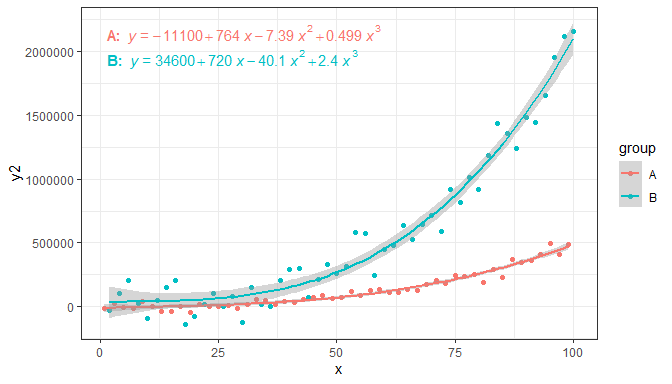Being able to label equations allows us to dispense with the use of color, which in many cases is needed for printed output.

``````formula <- y ~ poly(x, 3, raw = TRUE)
ggplot(my.data, aes(x, y2, linetype = group, grp.label = group)) +
geom_point() +
geom_smooth(method = "lm", formula = formula, color = "black") +
stat_poly_eq(aes(label = stat(paste("bold(", grp.label, "*':')~~~",
eq.label, sep = ""))),
formula = formula, parse = TRUE)``````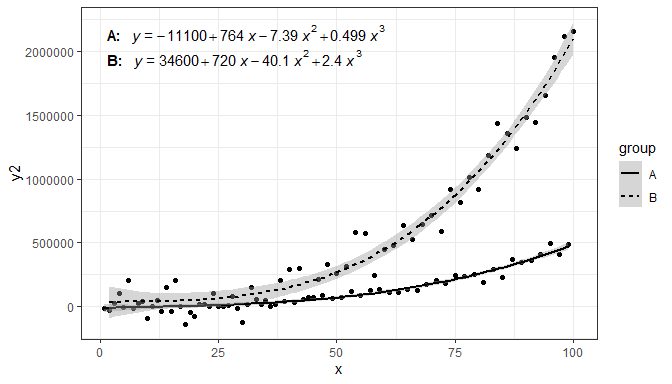Label positions relative to the ranges of the x and y scales are also supported, both through string constants and numeric values in the range 0 to 1, when using the default `geom_text_npc()`.

``````formula <- y ~ poly(x, 3, raw = TRUE)
ggplot(my.data, aes(x, y2, colour = group)) +
geom_point() +
geom_smooth(method = "lm", formula = formula) +
stat_poly_eq(aes(label = stat(eq.label)),
formula = formula, parse = TRUE,
label.x = "centre")``````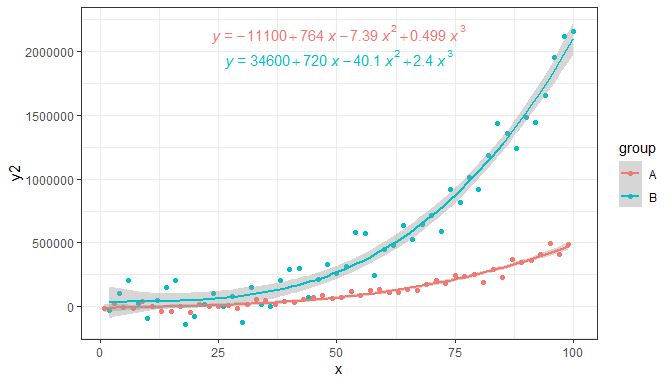The default locations are now based on normalized coordinates, and consequently these defaults work even when the range of the x and y scales varies from panel to panel.

``````formula <- y ~ poly(x, 3, raw = TRUE)
ggplot(my.data, aes(x, y2, fill = block)) +
geom_point(shape = 21, size = 3) +
geom_smooth(method = "lm", formula = formula) +
stat_poly_eq(aes(label = stat(rr.label)), size = 3,
geom = "label_npc", alpha = 0.33,
formula = formula, parse = TRUE) +
facet_wrap(~group, scales = "free_y")``````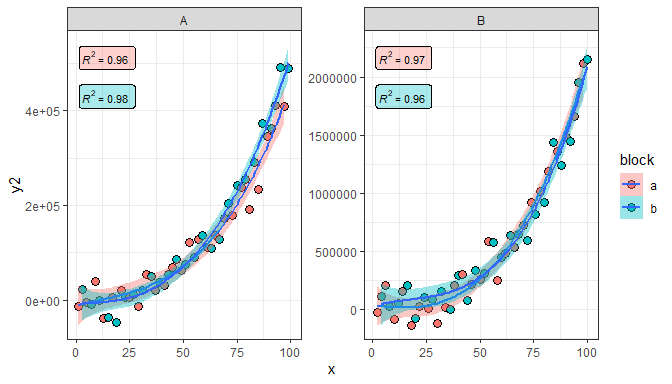If grouping is not the same within each panel created by faceting, the automatic location of labels results in “holes” for the factor levels not present in a given panel. In this case, consistent positioning requires passing explicitly the positions for each individual group. In the plot below the simultaneous mapping to both color and fill aesthetics creates four groups, two with data in panel A and the other two in panel B.

``````formula <- y ~ poly(x, 3, raw = TRUE)
ggplot(my.data, aes(x, y2, colour = group, fill = block)) +
geom_point(shape = 21, size = 3) +
geom_smooth(method = "lm", formula = formula) +
stat_poly_eq(aes(label = stat(rr.label)), size = 3, alpha = 0.2,
geom = "label_npc", label.y = c(0.95, 0.85, 0.95, 0.85),
formula = formula, parse = TRUE) +
facet_wrap(~group, scales = "free_y")``````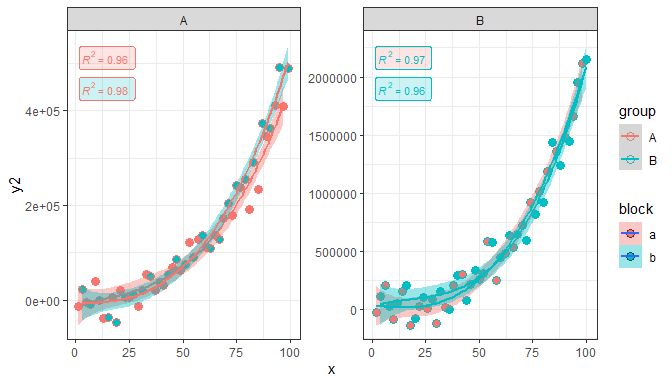It is possible to use `geom_text()` and `geom_label()` but in this case, if label coordinates are given explicitly they should be expressed in native data coordinates. When multiple labels need to be positioned a vector of coordinates can be used as shown here for `label.x` and `label.y`.

``````formula <- y ~ poly(x, 3, raw = TRUE)
ggplot(my.data, aes(x, y2, colour = group)) +
geom_point() +
geom_smooth(method = "lm", formula = formula) +
stat_poly_eq(geom = "text", aes(label = stat(eq.label)),
label.x = c(100, 90), label.y = c(-1e4, 2.1e6), hjust = "inward",
formula = formula, parse = TRUE)``````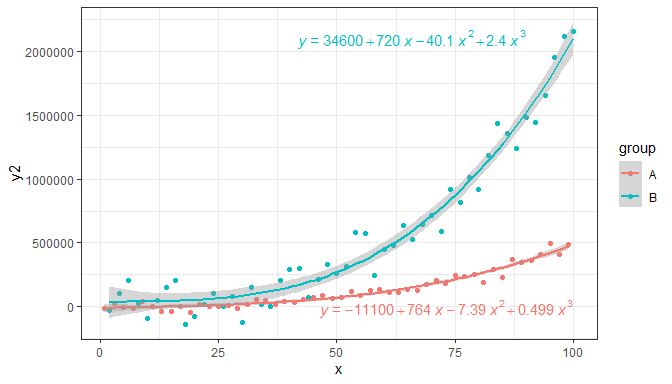Note: Automatic positioning using `geom_text()` and `geom_label()` is not supported when faceting uses free scales. In this case `geom_text_npc()` and/or `geom_label_npc()` must be used.

### stat_fit_residuals

I had the need to quickly plot residuals matching fits plotted with `geom_smooth()` using grouping and facets, so a new (simple) statistic was born.

``````formula <- y ~ poly(x, 3, raw = TRUE)
ggplot(my.data, aes(x, y, colour = group)) +
geom_hline(yintercept = 0, linetype = "dashed") +
stat_fit_residuals(formula = formula)``````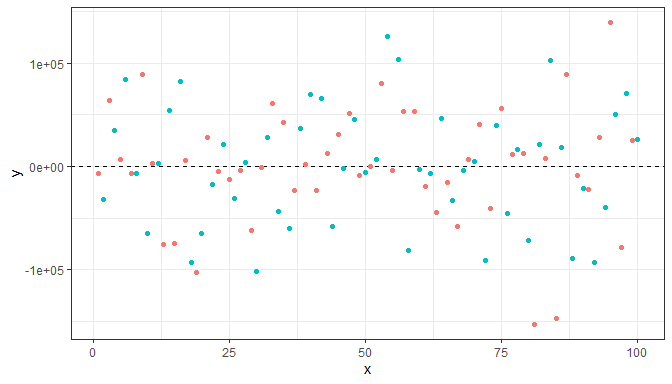### stat_fit_deviations

As needed to highlight residuals in slides and notes to be used in teaching, statistic `stat_fit_deviations` was born. It makes it easy to highlight the deviations of the fitted model from the individual observations.

``````formula <- y ~ poly(x, 3, raw = TRUE)
ggplot(my.data, aes(x, y)) +
geom_smooth(method = "lm", formula = formula) +
stat_fit_deviations(formula = formula, colour = "red") +
geom_point()``````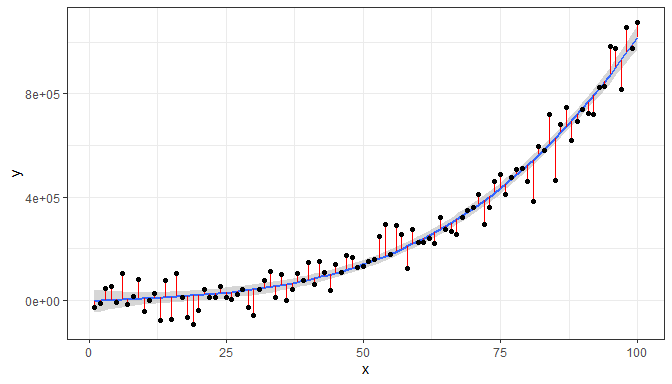The geometry used by default is `geom_segment()` to which additional aesthetics can be mapped. Here we add arrowheads.

``````formula <- y ~ poly(x, 3, raw = TRUE)
ggplot(my.data, aes(x, y)) +
geom_smooth(method = "lm", formula = formula) +
geom_point() +
stat_fit_deviations(formula = formula, colour = "red",
arrow = arrow(length = unit(0.015, "npc"),
ends = "both"))``````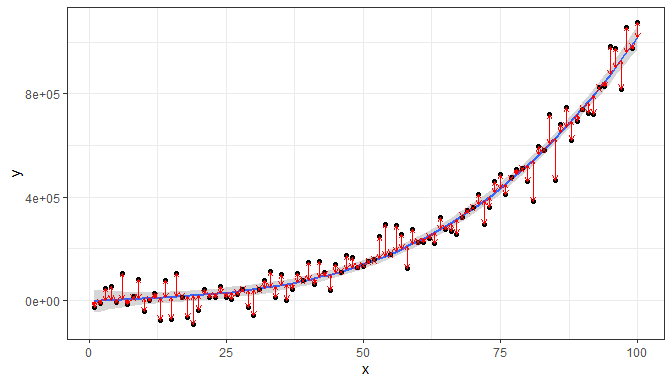### stat_fit_glance

Package ‘broom’ provides consistently formatted output from different model fitting functions. These made it possible to write a model-annotation statistic that is very flexible but that requires additional input from the user to generate the character strings to be mapped to the `label` aesthetic.

As we have above given some simple examples, we here exemplify this statistic in a plot with grouping, and assemble a label for the P-value using a string parsed into a expression. We also change the default position of the labels.

``````# formula <- y ~ poly(x, 3, raw = TRUE)
# broom::augment does not handle poly() correctly!
formula <- y ~ x + I(x^2) + I(x^3)
ggplot(my.data, aes(x, y, colour = group)) +
geom_point() +
geom_smooth(method = "lm", formula = formula) +
stat_fit_glance(method = "lm",
method.args = list(formula = formula),
label.x = "right",
label.y = "bottom",
aes(label = paste("italic(P)*\"-value = \"*",
signif(stat(p.value), digits = 4),
sep = "")),
parse = TRUE)``````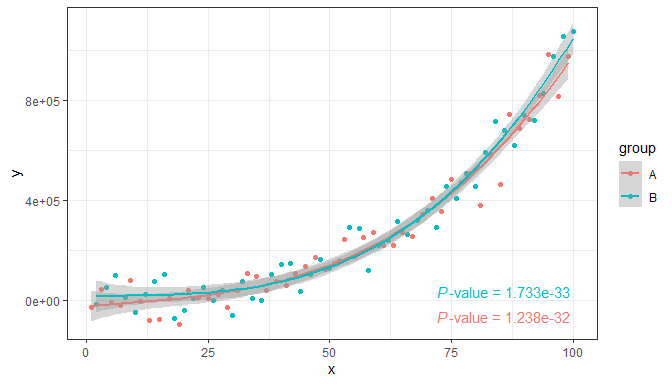It is also possible to fit a non-linear model with `method = "nls"`, and any other model for which a `glance()` method exists. Do consult the documentation for package ‘broom’. Here we fit the Michaelis-Menten equation to reaction rate versus concentration data.

``````micmen.formula <- y ~ SSmicmen(x, Vm, K)
ggplot(Puromycin, aes(conc, rate, colour = state)) +
geom_point() +
geom_smooth(method = "nls",
formula = micmen.formula,
se = FALSE) +
stat_fit_glance(method = "nls",
method.args = list(formula = micmen.formula),
aes(label = paste("AIC = ", signif(stat(AIC), digits = 3),
", BIC = ", signif(stat(BIC), digits = 3),
sep = "")),
label.x = "centre", label.y = "bottom")``````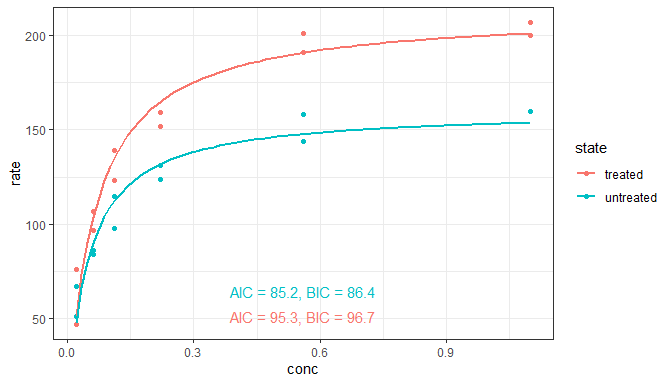### stat_fit_tidy

This stat makes it possible to add the equation for any fitted model for which `broom::tidy()` is implemented. Alternatively, individual values such as estimates for the fitted parameters, standard errors, or P-values can be added to a plot. However, the user has to explicitly construct the labels within `aes()`. This statistic respects grouping based on aesthetics, and reshapes the output of `tidy()` so that the values for a given group are in a single row in the returned `data`.

``````micmen.formula <- y ~ SSmicmen(x, Vm, K)
ggplot(Puromycin, aes(conc, rate, colour = state)) +
geom_point() +
geom_smooth(method = "nls",
formula = micmen.formula,
se = FALSE) +
stat_fit_tidy(method = "nls",
method.args = list(formula = micmen.formula),
label.x = "right",
label.y = "bottom",
aes(label = paste("V[m]~`=`~", signif(stat(Vm_estimate), digits = 3),
"%+-%", signif(stat(Vm_se), digits = 2),
"~~~~K~`=`~", signif(stat(K_estimate), digits = 3),
"%+-%", signif(stat(K_se), digits = 2),
sep = "")),
parse = TRUE)``````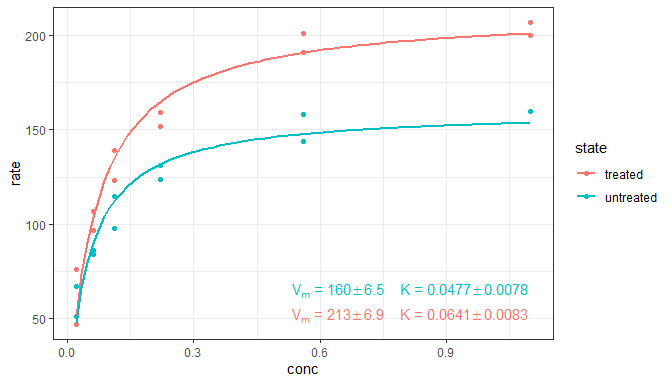Using paste we can build a string that can be parsed into an R expression, in this case for a non-linear equation.

``````micmen.formula <- y ~ SSmicmen(x, Vm, K)
ggplot(Puromycin, aes(conc, rate, colour = state)) +
geom_point() +
geom_smooth(method = "nls",
formula = micmen.formula,
se = FALSE) +
stat_fit_tidy(method = "nls",
method.args = list(formula = micmen.formula),
size = 3,
label.x = "center",
label.y = "bottom",
vstep = 0.18,
aes(label = paste("V~`=`~frac(", signif(stat(Vm_estimate), digits = 2), "~C,",
signif(stat(K_estimate), digits = 2), "+C)",
sep = "")),
parse = TRUE) +
labs(x = "C", y = "V")``````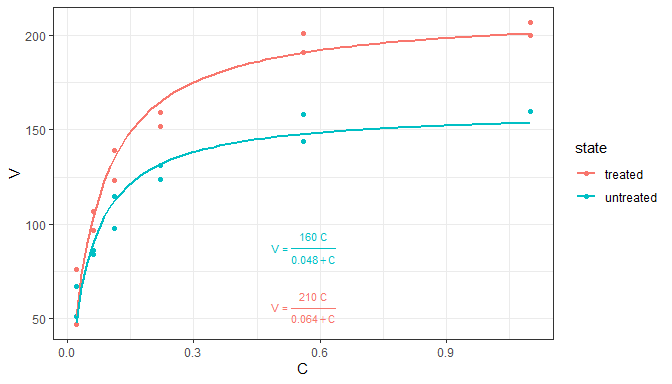### stat_fit_tb

This stat makes it possible to add summary or ANOVA tables for any fitted model for which `broom::tidy()` is implemented. The output from `tidy()` is embedded as a single list value within the returned `data`, an object of class `tibble`. This statistic ignores grouping based on aesthetics. This allows fitting models when `x` or `y` is a factor (as in such cases `ggplot` splits the data into groups, one for each level of the factor, which is needed for example for `stat_summary()` to work as expected). By default, the `"table"` geometry is used. The use of `geom_table()` is described in a separate section of this User Guide. Table themes and mapped aesthetics are supported.

The default output of `stat_fit_tb` is the default output from `tidy(mf)` where `mf` is the fitted model.

``````formula <- y ~ x + I(x^2) + I(x^3)
ggplot(my.data, aes(x, y)) +
geom_point() +
geom_smooth(method = "lm", formula = formula) +
stat_fit_tb(method = "lm",
method.args = list(formula = formula),
tb.vars = c(Parameter = "term",
Estimate = "estimate",
"s.e." = "std.error",
"italic(t)" = "statistic",
"italic(P)" = "p.value"),
label.y = "top", label.x = "left",
parse = TRUE)``````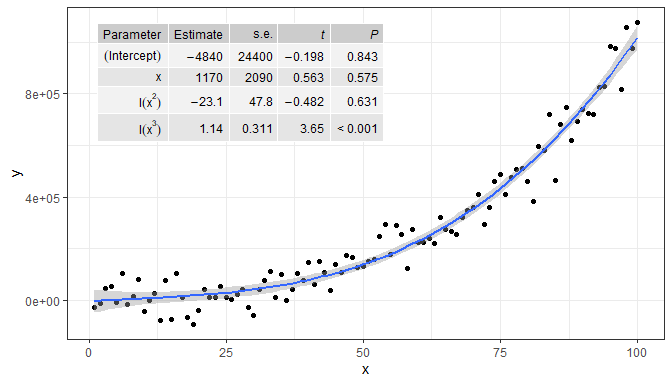When `tb.type = "fit.anova"` the output returned is that from `tidy(anova(mf))` where `mf` is the fitted model. Here we also show how to replace names of columns and terms, and exclude one column, in this case, the mean squares.

``````formula <- y ~ x + I(x^2) + I(x^3)
ggplot(my.data, aes(x, y)) +
geom_point() +
geom_smooth(method = "lm", formula = formula) +
stat_fit_tb(method = "lm",
method.args = list(formula = formula),
tb.type = "fit.anova",
tb.vars = c(Effect = "term",
df = "df",
"italic(F)" = "statistic",
"italic(P)" = "p.value"),
tb.params = c(x = 1, "x^2" = 2, "x^3" = 3, Resid = 4),
label.y = "top", label.x = "left",
parse = TRUE)``````
``## Dropping column(s) from table.``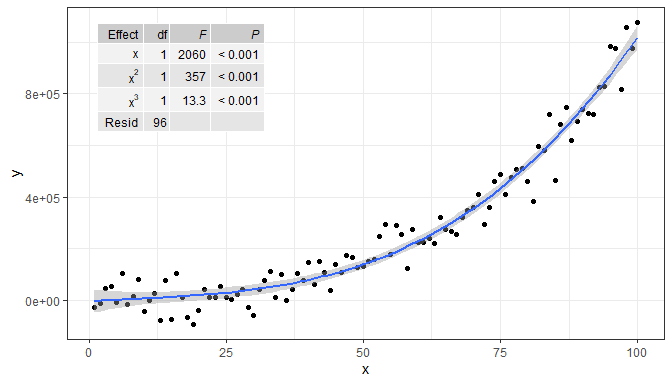When `tb.type = "fit.coefs"` the output returned is that of `tidy(mf)` after selecting the `term` and `estimate` columns.

``````formula <- y ~ x + I(x^2) + I(x^3)
ggplot(my.data, aes(x, y)) +
geom_point() +
geom_smooth(method = "lm", formula = formula) +
stat_fit_tb(method = "lm",
method.args = list(formula = formula),
tb.type = "fit.coefs",
label.y = "center", label.x = "left")``````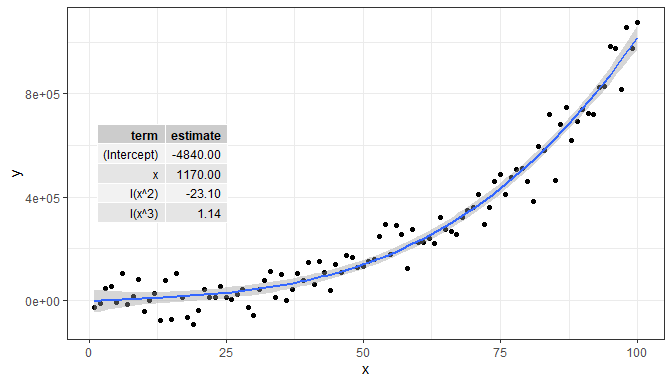Faceting works as expected, but grouping is ignored as mentioned above. In this case, the color aesthetic is not applied to the text of the tables. Furthermore, if `label.x.npc` or `label.y.npc` are passed numeric vectors of length > 1, the corresponding values are obeyed by the different panels.

``````micmen.formula <- y ~ SSmicmen(x, Vm, K)
ggplot(Puromycin, aes(conc, rate, colour = state)) +
facet_wrap(~state) +
geom_point() +
geom_smooth(method = "nls",
formula = micmen.formula,
se = FALSE) +
stat_fit_tb(method = "nls",
method.args = list(formula = micmen.formula),
tb.type = "fit.coefs",
label.x = 0.9,
label.y = c(0.75, 0.2)) +
theme(legend.position = "none") +
labs(x = "C", y = "V")``````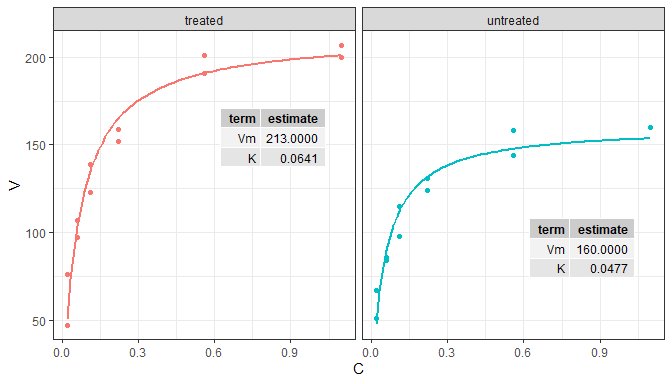The data in the example below are split by `ggplot` into six groups based on the levels of the `feed` factor. However, as `stat_fit_tb()` ignores groupings, we can still fit a linear model to all the data in the panel.

``````ggplot(chickwts, aes(factor(feed), weight)) +
stat_summary(fun.data = "mean_se") +
stat_fit_tb(tb.type = "fit.anova",
label.x = "center",
label.y = "bottom") +
expand_limits(y = 0)``````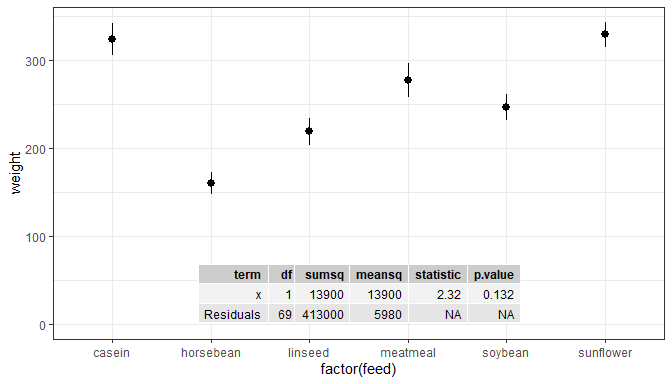We can flip the system of coordinates, if desired.

``````ggplot(chickwts, aes(factor(feed), weight)) +
stat_summary(fun.data = "mean_se") +
stat_fit_tb(tb.type = "fit.anova", label.x = "left", size = 3) +
scale_x_discrete(expand = expansion(mult = c(0.2, 0.5))) +
coord_flip()``````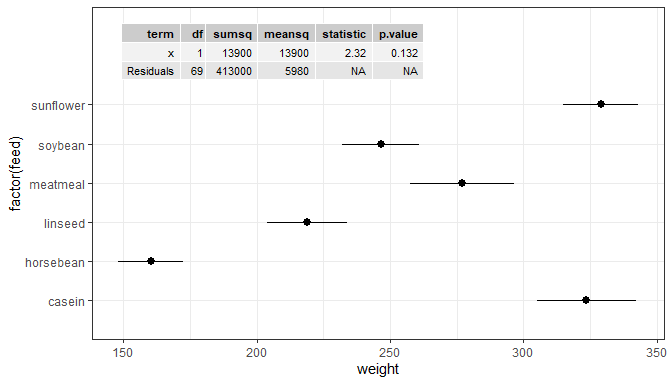It is also possible to rotate the table using `angle`. Here we also show how to replace the column headers with strings to be parsed into R expressions.

``````ggplot(chickwts, aes(factor(feed), weight)) +
stat_summary(fun.data = "mean_se") +
stat_fit_tb(tb.type = "fit.anova",
angle = 90, size = 3,
label.x = "right", label.y = "center",
hjust = 0.5, vjust = 0,
tb.vars = c(Effect = "term",
"df",
"M.S." = "meansq",
"italic(F)" = "statistic",
"italic(P)" = "p.value"),
parse = TRUE) +
scale_x_discrete(expand = expansion(mult = c(0.1, 0.35))) +
expand_limits(y = 0)``````
``## Dropping column(s) from table.``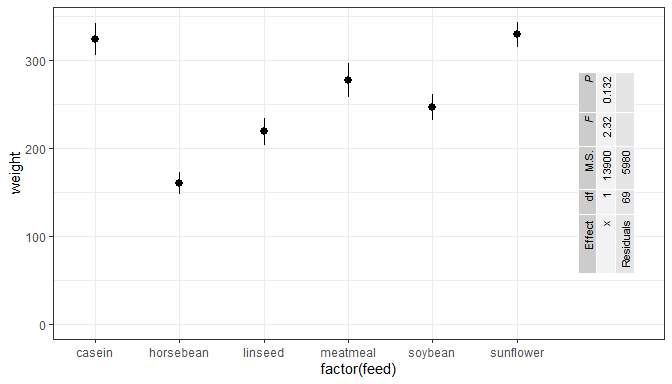### stat_fit_augment

Experimental! Use `ggplot2::stat_smooth` instead of `stat_fit_augment` if possible.

For a single panel and no grouping, there is little advantage in using this statistic compared to the examples in the documentation of package ‘broom’. With grouping and faceting `stat_fit_augment` may occasionally be more convenient than `ggplot2::stat_smooth` because of its flexibility.

``````# formula <- y ~ poly(x, 3, raw = TRUE)
# broom::augment does not handle poly correctly!
formula <- y ~ x + I(x^2) + I(x^3)
ggplot(my.data, aes(x, y)) +
geom_point() +
stat_fit_augment(method = "lm",
method.args = list(formula = formula))``````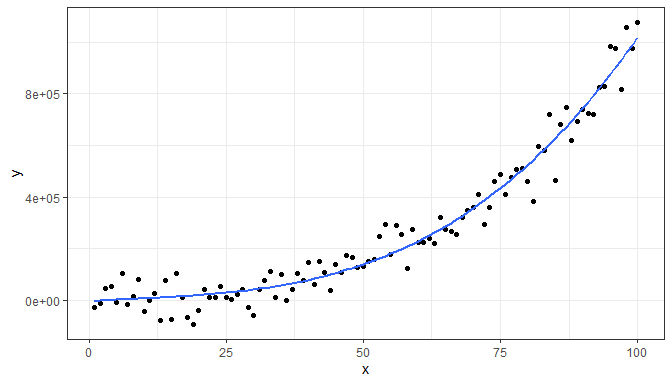``````formula <- y ~ x + I(x^2) + I(x^3)
ggplot(my.data, aes(x, y, colour = group)) +
geom_point() +
stat_fit_augment(method = "lm",
method.args = list(formula = formula))``````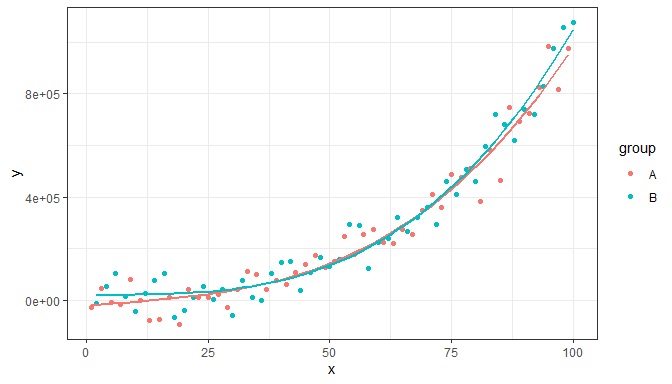We can override the variable returned as `y` to be any of the variables in the data frame returned by `broom::augment` while still preserving the original `y` values.

``````formula <- y ~ x + I(x^2) + I(x^3)
ggplot(my.data, aes(x, y)) +
stat_fit_augment(method = "lm",
method.args = list(formula = formula),
geom = "point",
y.out = ".resid")``````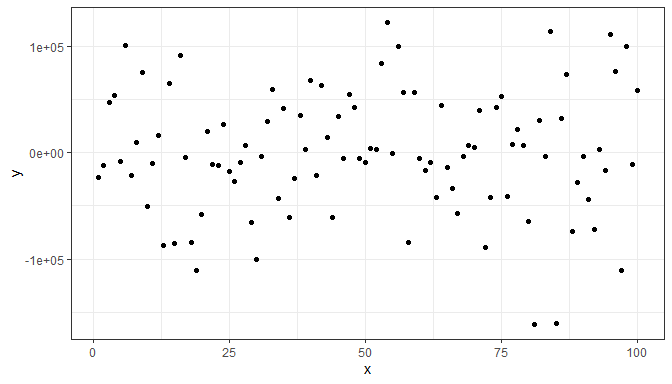``````formula <- y ~ x + I(x^2) + I(x^3)
ggplot(my.data, aes(x, y, colour = group)) +
stat_fit_augment(method = "lm",
method.args = list(formula = formula),
geom = "point",
y.out = ".std.resid")``````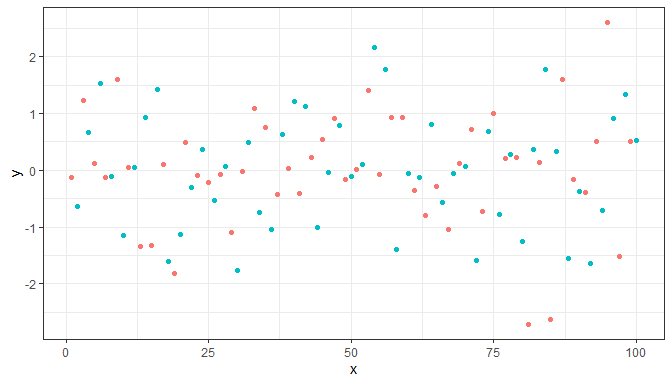We can use any model fitting method for which `augment` is implemented.

``````args <- list(formula = y ~ k * e ^ x,
start = list(k = 1, e = 2))
ggplot(mtcars, aes(wt, mpg)) +
geom_point() +
stat_fit_augment(method = "nls",
method.args = args)``````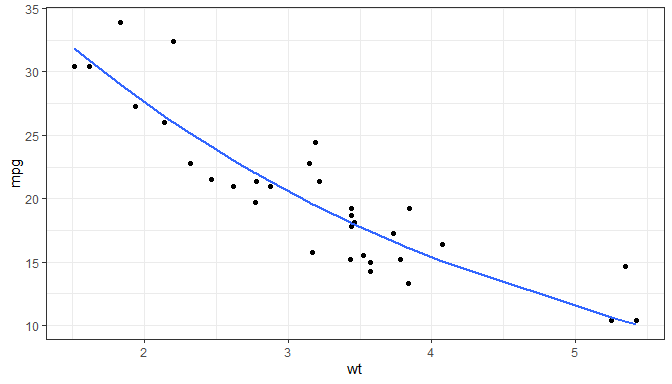``````args <- list(formula = y ~ k * e ^ x,
start = list(k = 1, e = 2))
ggplot(mtcars, aes(wt, mpg)) +
stat_fit_augment(method = "nls",
method.args = args,
geom = "point",
y.out = ".resid")``````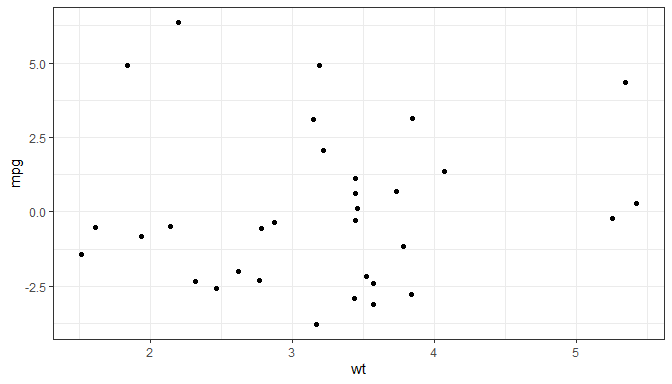### stat_apply_group and stat_apply_panel

These statistics apply a function to `x` and `y` data. The function is expected to return a vector of the same length as its input. It is usually better to apply such functions through mappings using `aes()` when they are independent of grouping or to use a transformation for the scale. However, when grouping is important, these statistics solve a problem that would otherwise require pre-computation of the data. Here we plot the difference in circumference between dates for each tree. (As the vector returned by `diff()` is one element shorter than its input, we prepend `NA`.)

``````ggplot(Orange, aes(age, circumference, colour = Tree)) +
stat_apply_group(.fun.y = function(x) {c(NA, diff(x))}, na.rm = TRUE)``````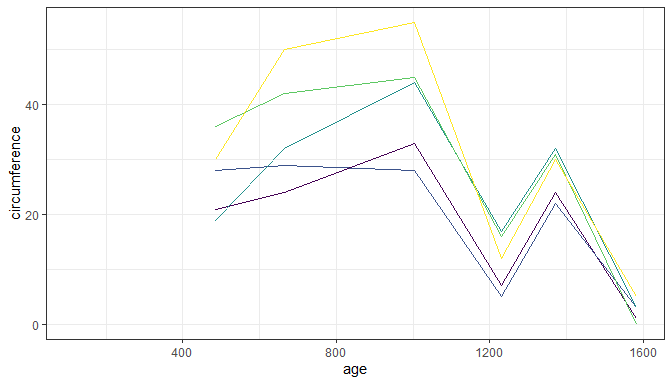### stat_dens2d_labels and stat_dens2d_filter

These stats had their origin in an enhancement suggestion for ‘ggrepel’ from Hadley Wickham and discussion with Kamil Slowikowski (ggrepel’s author) and others. In fact the code is based on code Kamil gave during the discussion, but simplified and taking a few further ideas from `ggplot::stat_dens2d`.

Warning! Which observations are selected by the algorithm used, based on `MASS:kde2d`, depends strongly on the values of parameters `h` and `n`. You may need to alter the defaults by passing explicit arguments to these stats. Beware, though, that what are good values, may depend on individual data sets even if they include the same number of observations. For the selection of observations to work cleanly, the argument for `n` must create a dense grid. Much larger values of `n` than in the examples in the documentation of `MASS::kde2d` and `ggplot2::stat_dens2d` will be needed in most cases.

Some random data with random labels.

``````random_string <- function(len = 3) {
paste(sample(letters, len, replace = TRUE), collapse = "")
}

# Make random data.
set.seed(1001)
d <- tibble::tibble(
x = rnorm(100),
y = rnorm(100),
group = rep(c("A", "B"), c(50, 50)),
lab = replicate(100, { random_string() })
)``````

### stat_dens2d_filter

The stat `stat_dens2d_filter` filters observations, in other words passes to the geom a subset of the data received as input. The default argument for `geom` is `"point"`.

Using defaults except for the color aesthetic. Highlight 1/4 of observations from lowest density areas of the plot panel.

``````ggplot(data = d, aes(x, y)) +
geom_point() +
stat_dens2d_filter(keep.fraction = 1/4, colour = "red")``````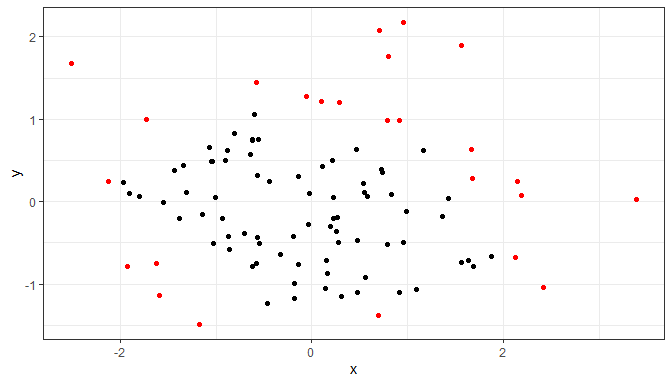Keep at most 20 observations.

``````ggplot(data = d, aes(x, y)) +
geom_point() +
stat_dens2d_filter(keep.fraction = 1/4, keep.number = 50, colour = "red")``````Keep always 50 observations.

``````ggplot(data = d, aes(x, y)) +
geom_point() +
stat_dens2d_filter(keep.fraction = 1, keep.number = 50, colour = "red")``````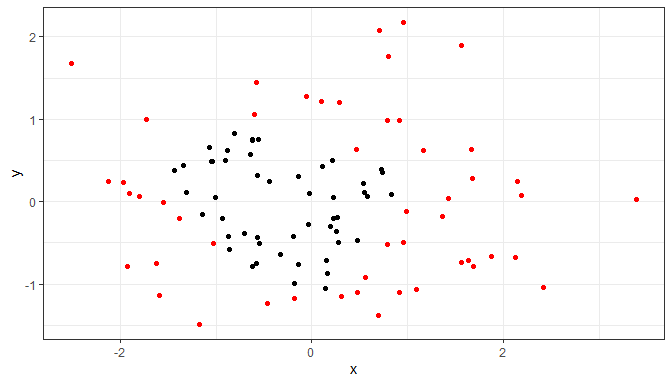Highlighting 1/4 of the observations by under-plotting with larger black points.

``````ggplot(data = d, aes(x, y, colour = group)) +
stat_dens2d_filter(keep.fraction = 0.25,
size = 3,
colour = "black") +
geom_point()``````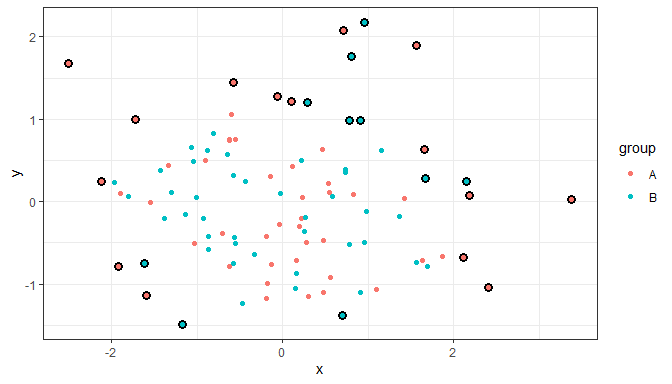A different way of highlighting 1/4 of the observations, using over-plotting with a ‘hollow’ shape. We also shift one group with respect to the other.

``````ggplot(data = d, aes(x + rep(c(-2,2), rep(50,2)),
y, colour = group)) +
geom_point() +
stat_dens2d_filter(shape = 1, size = 3,
keep.fraction = 0.25)``````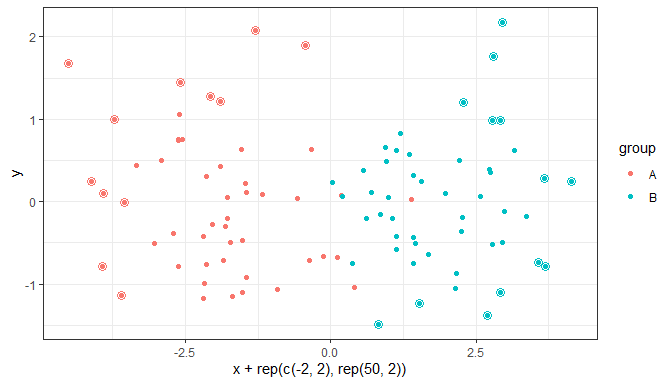Highlight 1/4 of observations from lowest density areas of the plot, with density considered separately for each individual group. In this case grouping is based on the color aesthetic.

``````ggplot(data = d, aes(x + rep(c(-2,2), rep(50,2)),
y, colour = group)) +
geom_point() +
stat_dens2d_filter_g(shape = 1, size = 3,
keep.fraction = 0.25)``````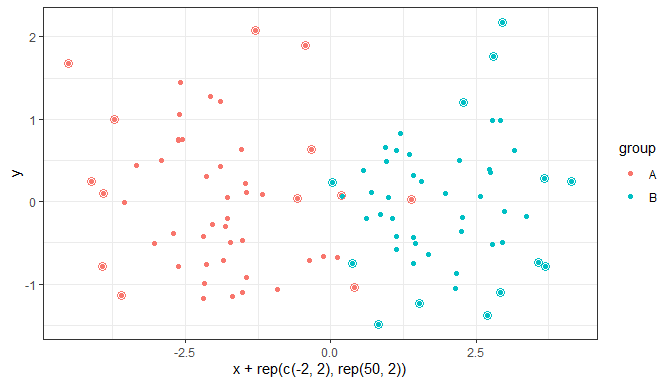### stat_dens2d_labels

The stat `stat_dens1d_labels` replaces the values of the label (aesthetic) variable in `data` based on density of observations along the x or y axis in the plot panel. The replacement is given by the argument passed to `label.fill`, which can be a character string or a function accepting a character string as argument and returning also a character string.

The default value for `geom` is `"text"`. The default value of `label.fill` is `""` which results in empty labels, while using `NA` as fill label results in observations being omitted. Using `NA` as `label.fill` is similar to using `stat_dens2d_filter` as long as the geom used requires a `label` aesthetic.

Label 1/10 of observations from lowest density areas of the plot panels.

``````ggplot(data = d, aes(x, y, label = lab, colour = group)) +
stat_dens2d_labels(keep.fraction = 1/10,
hjust = "outward", vjust = "outward") +
geom_point()``````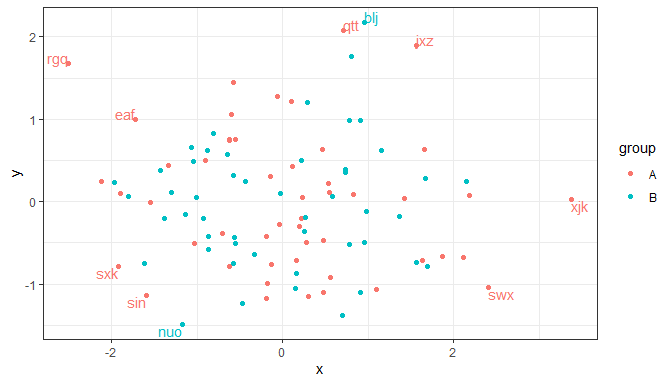Using the geoms from package ‘ggrepel’ avoids clashes among labels or on top of data points. This works with versions 0.6.0 and newer of ‘ggrepel’. One example with `geom_text_repel` follows.

``````ggplot(data = d, aes(x, y, label = lab, colour = group)) +
geom_point() +
stat_dens2d_labels(geom = "text_repel",
keep.fraction = 0.45)``````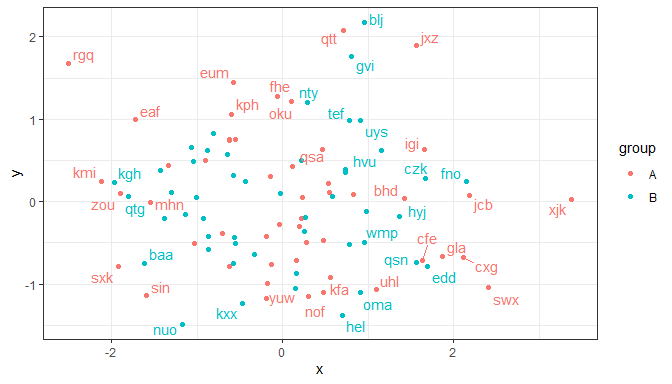With `geom_label_repel` one usually needs to use a smaller value for `keep.fracton`, or a smaller `size`, as labels use more space on the plot than the test alone.

Additional arguments can be used to change the angle and position of the text, but may give unexpected output when labels are long as the repulsion algorithm “sees” always a rectangular bounding box that is not rotated. With short labels or angles that are multiples of 90 degrees, there is no such problem. Please, see the documentation for `ggrepel::geom_text_repel` and `ggrepel::geom_label_repel` for the various ways in which both repulsion and formatting of the labels can be adjusted.

Using `NA` as argument to `label.fill` makes the observations with labels set to `NA` incomplete, and such rows in data are skipped when rendering the plot, before the repulsion algorithm is active. This can lead to overlap between text and points corresponding to unlabelled observations. Whether points are occluded depends on the order of layers and transparency, the occlusion can remain easily unnoticed with `geom_label` and `geom_label_repel`. We keep `geom_point` as the topmost layer to ensure that all observations are visible.

``````ggplot(data = d, aes(x, y, label = lab, colour = group)) +
stat_dens2d_labels(geom = "label_repel",
keep.fraction = 0.35,
label.fill = NA) +
geom_point()``````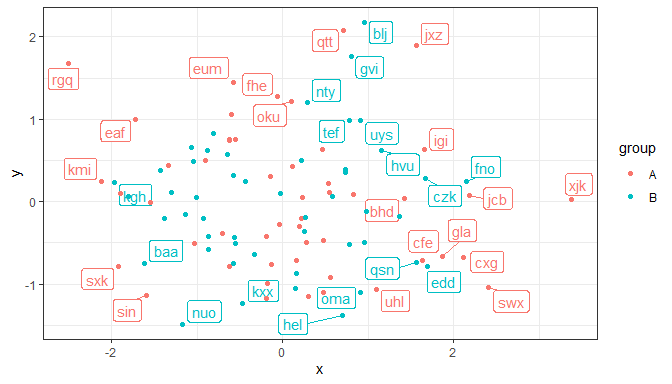### stat_dens1d_labels and stat_dens1d_filter

These stats are similar to `stat_dens2d_labels()` and `stat_dens2d_filter()` but compute the density in a single dimension, either the x or y aesthetics.

Warning! Which observations are selected by the algorithm used, based on `stats::density`, depends strongly on the values of parameters `bw`, `adjust` and `kernel`. You may need to alter the defaults by passing explicit arguments. Beware that what are good values, may depend on individual data sets even if they include the same number of observations. For the selection of observations to work cleanly, the argument for `n` must large enough to generate a dense grid or the bandwidth may need to be increased by passing a number > 1 as argument. Increasing the bandwidth makes the empirical density function smoother, and the selection of points less dependent on immediate neighbors.

We use the same data as in the previous sections.

``````random_string <- function(len = 6) {
paste(sample(letters, len, replace = TRUE), collapse = "")
}

# Make random data.
set.seed(1001)
d <- tibble::tibble(
x = rnorm(100),
y = rnorm(100),
group = rep(c("A", "B"), c(50, 50)),
lab = replicate(100, { random_string() })
)``````

### stat_dens1d_filter

The stat `stat_dens1d_filter` filters observations, in other words passes to the geom a subset of the data received as input. The default value for `geom` is `"point"` and the default `orientation` is `"x"`.

Using defaults except for the color aesthetic, we highlight 1/4 of observations from lowest density region along the x axis of the plot panel.

``````ggplot(data = d, aes(x, y)) +
geom_point() +
stat_dens1d_filter(keep.fraction = 0.25,
colour = "red")``````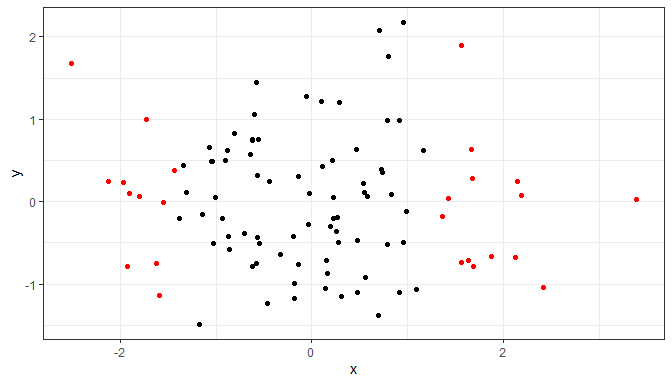We repeat the example above, we highlight 1/4 of observations, but now from lowest density region along the y axis of the plot panel.

``````ggplot(data = d, aes(x, y)) +
geom_point() +
stat_dens1d_filter(keep.fraction = 0.25,
colour = "red",
orientation = "y")``````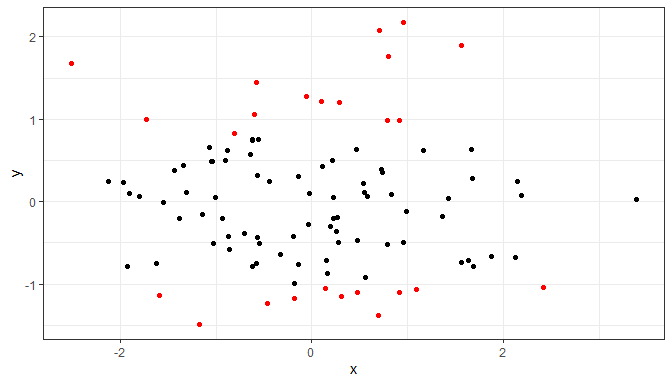In other respects than `orientation` and the parameters passed internally to `stats::density()` the examples given earlier for `stat_dens2d_filter()` also apply.

### stat_dens1d_labels

The stat `stat_dens1d_labels` replaces the values of the label (aesthetic) variable in `data` based on density of observations along the x or y axis in the plot panel. The replacement is given by the argument passed to `label.fill`, which can be a character string or a function accepting a character string as argument and returning also a character string.

The default value for `geom` is `"text"`. The default value of `label.fill` is `""` which results in empty labels, while using `NA` as fill label results in observations being omitted. Using `NA` as `label.fill` is similar to using `stat_dens2d_filter` as long as the geom used requires a `label` aesthetic.

Label 1/10 of observations from lowest density regions along x in the plot panel. Normally a repulsive geom is most useful.

``````ggplot(data = d, aes(x, y, label = lab, colour = group)) +
geom_point() +
stat_dens1d_labels(geom = "text_repel")``````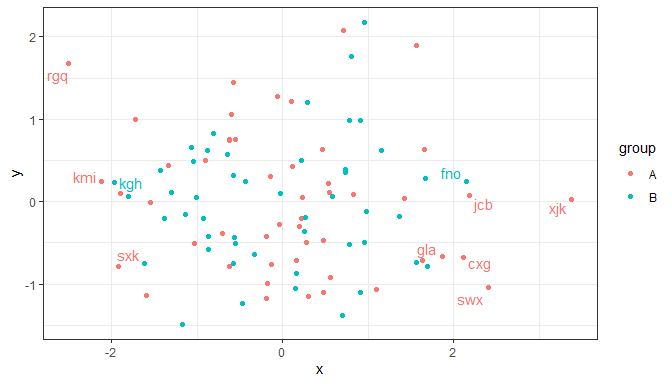Similarly along the y axis.

``````ggplot(data = d, aes(x, y, label = lab, colour = group)) +
geom_point() +
stat_dens1d_labels(geom = "text_repel", orientation = "y")``````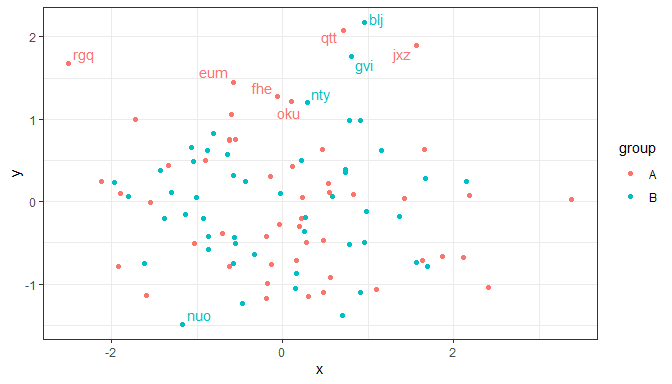In other respects than `orientation` and the parameters passed internally to `stats::density()` the examples given earlier for `stat_dens2d_labels()` also apply.

## Scales

Volcano and quadrant plots are scatter plots with some peculiarities. Creating these plots from scratch using ‘ggplot2’ can be time consuming, but allows flexibility in design. Rather than providing a ‘canned’ function to produce volcano plots, package ‘ggpmisc’ provides several building blocks that facilitate the production of volcano and quadrant plots as wrappers on scales with suitable defaults plus helper functions to create factors from numeric outcomes.

### scale_color_outcome, scale_fill_outcome

Manual scales for color and fill aesthetics with defaults suitable for the three way outcomes from statistical tests.

### scale_x_FDR, scale_y_FDR, scale_x_logFC, scale_y_logFC, scale_x_Pvalue, scale_y_Pvalue

Scales for x or y aesthetics mapped to P-values, FDR (false discovery rate) or log FC (logarithm of fold-change) as used in volcano and quadrant plots of transcriptomics and metabolomics data.

### symmetric_limits

A simple function to expand scale limits to be symmetric around zero. Can be passed as argument to parameter limits of continuous scales from packages ‘ggpmisc’, ‘ggplot2’ or ‘scales’ (and extensions).

### Volcano-plot examples

Volcano plots are frequently used for transcriptomics and metabolomics. They are used to show P-values or FDR (false discovery rate) as a function of effect size, which can be either an increase or a decrease. Effect sizes are expressed as fold-changes compared to a control or reference condition. Colors are frequently used to highlight significant responses. Counts of significant increases and decreases are frequently also added.

Outcomes encoded as -1, 0 or 1, as seen in the tibble below need to be converted into factors with suitable labels for levels. This can be easily achieved with function `outcome2factor()`.

``head(volcano_example.df) ``
``````##         tag     gene outcome       logFC     PValue genotype
## 1 AT1G01040     ASU1       0 -0.15284466 0.35266997      Ler
## 2 AT1G01290     ASG4       0 -0.30057068 0.05471732      Ler
## 3 AT1G01560 ATSBT1.1       0 -0.57783350 0.06681310      Ler
## 4 AT1G01790   AtSAM1       0 -0.04729662 0.74054263      Ler
## 5 AT1G02130  AtTRM82       0 -0.14279891 0.29597519      Ler
## 6 AT1G02560    PRP39       0  0.23320752 0.07487043      Ler``````

Most frequently fold-change data is available log-transformed, using either 2 or 10 as base. In general it is more informative to use tick labels expressed as un-transformed fold-change.

By default `scale_x_logFC()` and `scale_y_logFC()` expect the logFC data log2-transformed, but this can be overridden. The default use of untransformed fold-change for tick labels can also be overridden. Scale `scale_x_logFC()` in addition by default expands the scale limits to make them symmetric around zero. If `%unit` is included in the name character string, suitable units are appended as shown in the example below.

Scales `scale_y_Pvalue()` and `scale_x_Pvalue()` have suitable defaults for name and labels, while `scale_colour_outcome()` provides suitable defaults for the colors mapped to the outcomes. To change the labels and title of the `key` or `guide` pass suitable arguments to parameters `name` and `labels` of these scales. These x and y scales by default squish off-limits (out-of-bounds) observations towards the limits.

``````volcano_example.df %>%
mutate(., outcome.fct = outcome2factor(outcome)) %>%
ggplot(., aes(logFC, PValue, colour = outcome.fct)) +
geom_point() +
scale_x_logFC(name = "Transcript abundance%unit") +
scale_y_Pvalue() +
scale_colour_outcome() +
stat_quadrant_counts(data = . %>% filter(outcome != 0))``````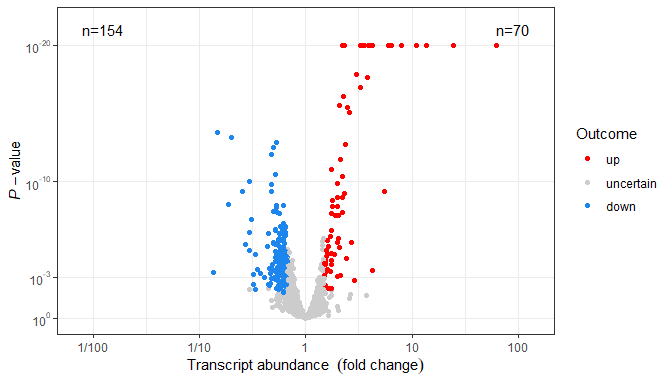By default `outcome2factor()` creates a factor with three levels as in the example above, but this default can be overridden as shown below.

``````volcano_example.df %>%
mutate(., outcome.fct = outcome2factor(outcome, n.levels = 2)) %>%
ggplot(., aes(logFC, PValue, colour = outcome.fct)) +
geom_point() +
scale_x_logFC(name = "Transcript abundance%unit") +
scale_y_Pvalue() +
scale_colour_outcome() +
stat_quadrant_counts(data = . %>% filter(outcome != 0))``````